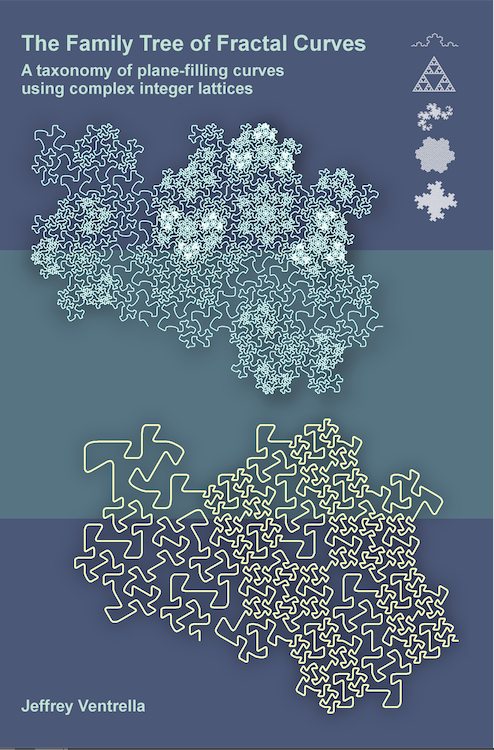The Family Tree of Fractal Curves A taxonomy of plane-filling curves using complex integer lattices by Jeffrey Ventrella 1 Introduction 2 Taxonomy 3 Iteration 4 Morphology 5 Family Resemblances 6 Undiscovered Curiosities 7 References

 4. Morphology For fractal enthusiasts, the morphological similarities and differences among fractals invites curiosity and wonder. Let’s explore the emergent attributes of plane-filling curves, with consideration to how they relate to the concepts covered thus far.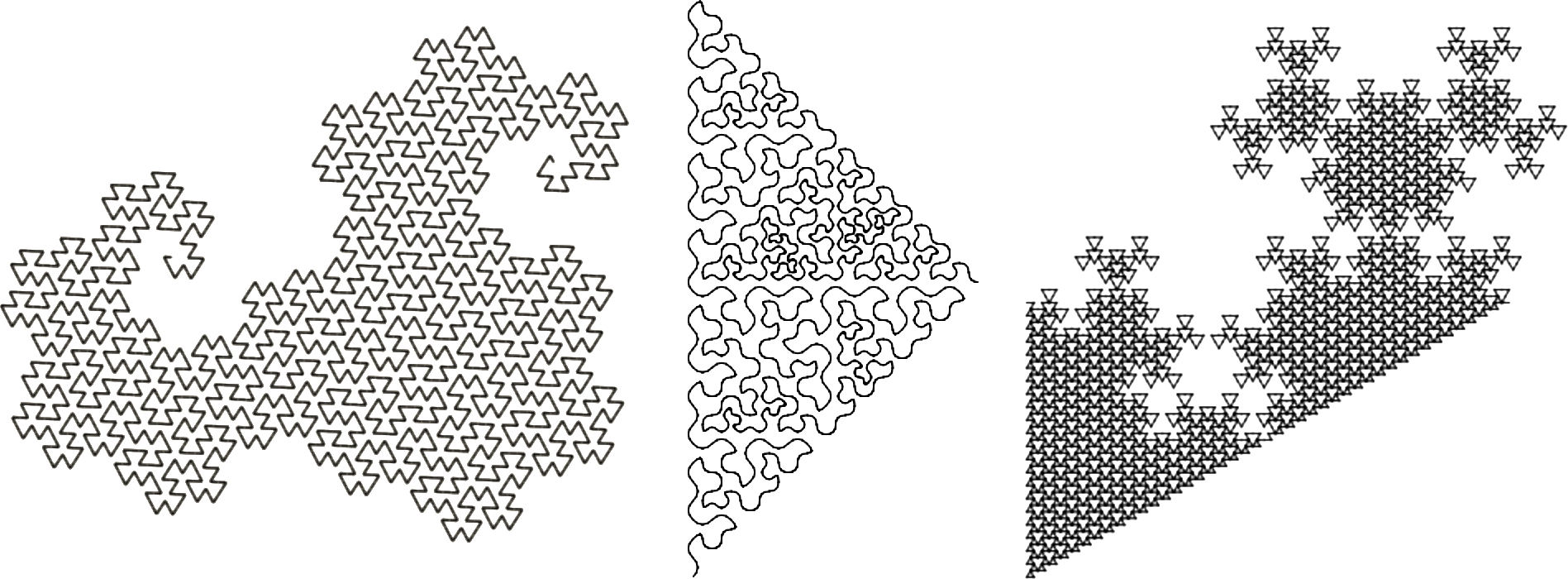Figure 4.1. Curves from the E(3,1), G(2,2), and E(2,0) families Mandelbrot suggested that fractals not only stimulate our aesthetic senses; they also serve as models of nature. He used ample illustrations in his introductions of fractals. Mandelbrot was a mathematician working in a discipline that has traditionally been skeptical of visual illustrations, often considering them poor substitutes for (or distractions from) pure mathematical ideas. This may be true for trained experts with exceptional talents in abstraction…with enough internal processing power to visualize an equation and bring it to life—in the mind’s eye. It is also true that a poorly designed illustration can cause ambiguity or distraction. On the other hand, a well-crafted picture is not only worth a thousand words; it also can stimulate the imagination—which motivates understanding, or at least curiosity. With that in mind, let’s introduce some visual metaphors from nature.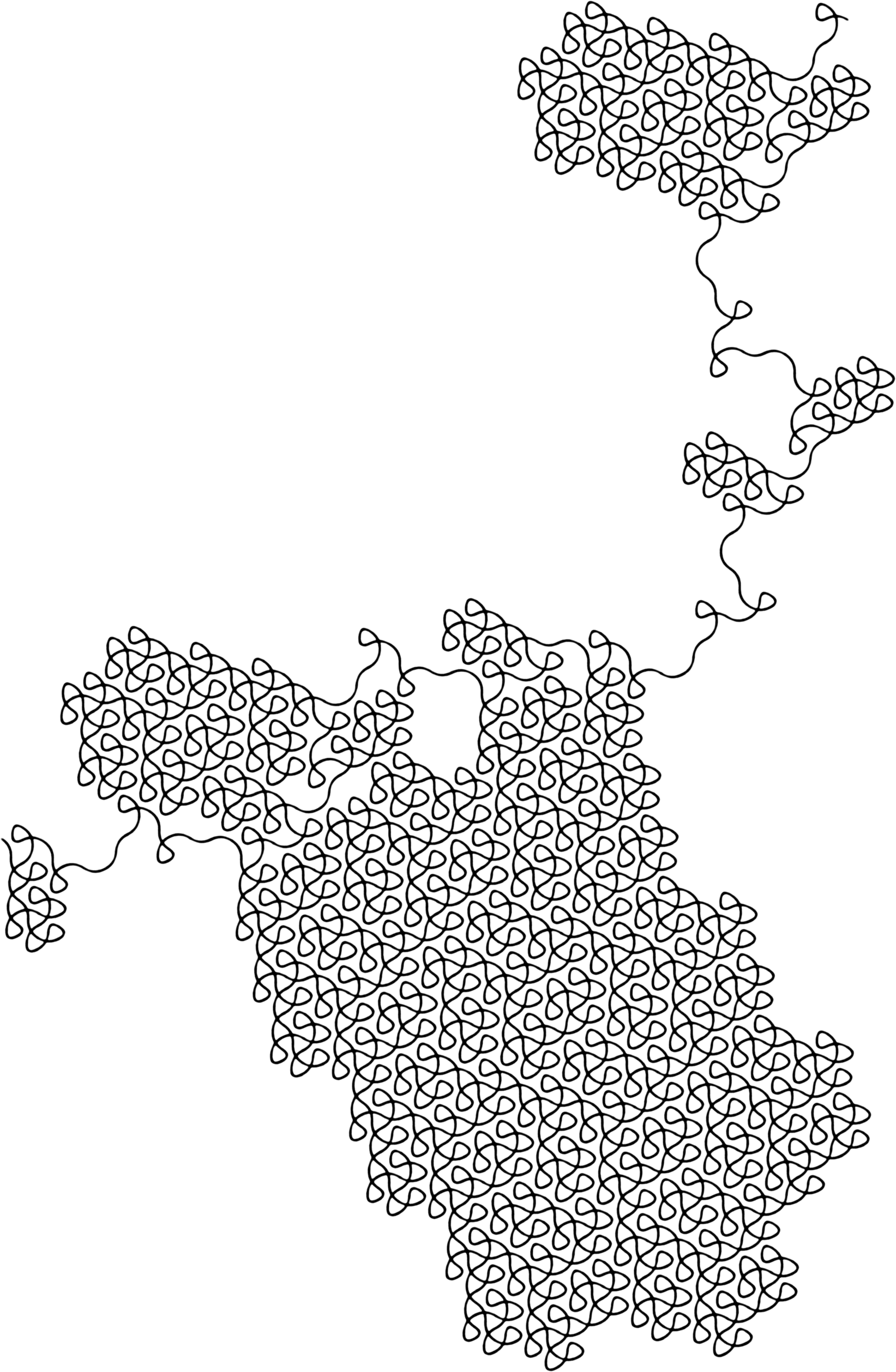Figure 4.2. A self-crossing curve from the E(3,1) family (splined) Spine, Flesh, Skin Like living organisms, fractal curves can only come into existence through a process of iteration. And, like living organisms, the process of growth results in forms that become increasingly complex.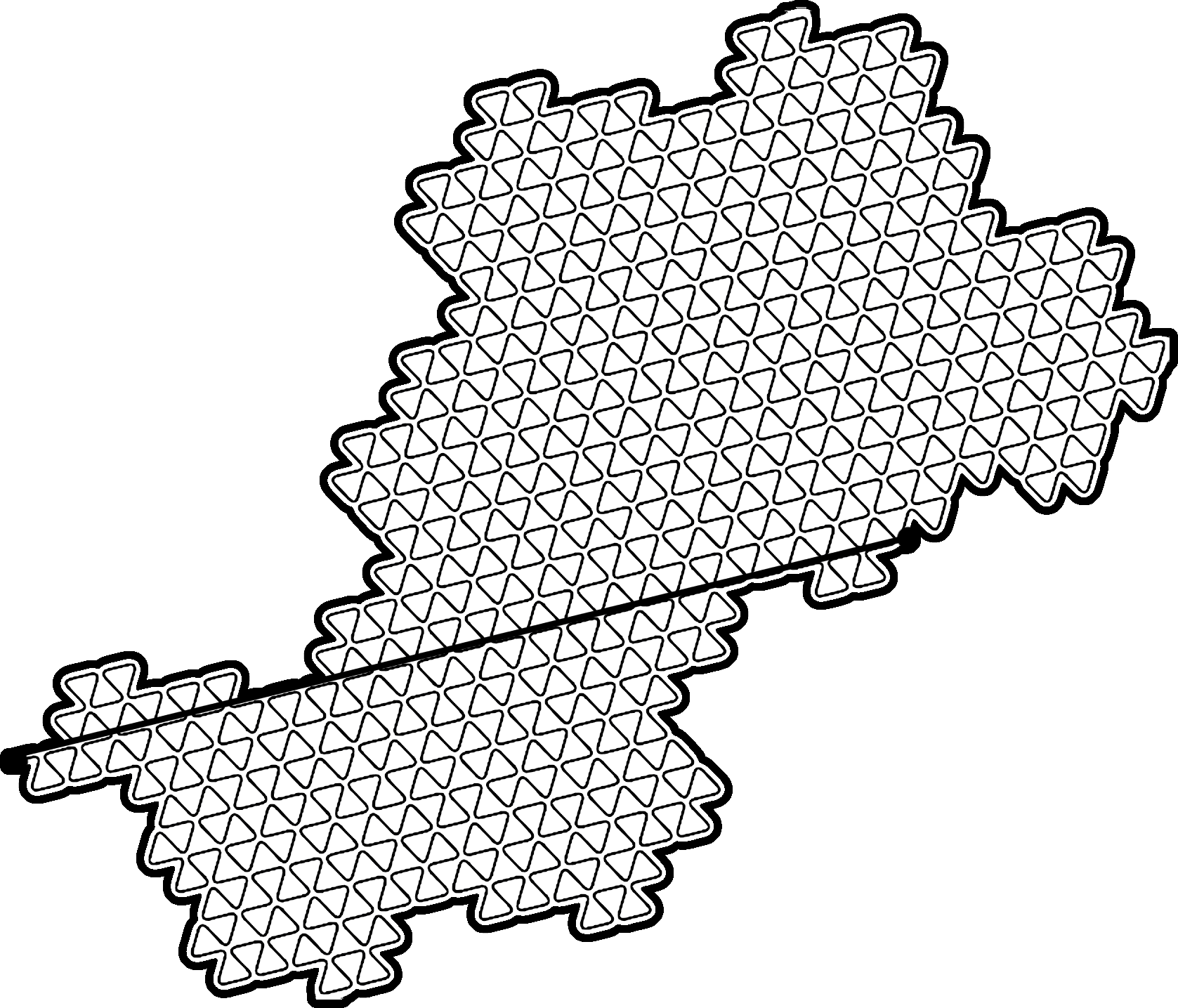Figure 4.3. Spine, flesh and skin—shown in a curve of the E(3,1) family Spine Every family can be represented by a vector that extends from the origin to the family integer. “Spine” is a poetic way of referring to the family integer, visualized as a 2D vector. All curves of a common family share a common spine, and each family spine is unique. Figure 4.4 shows some examples in both domains.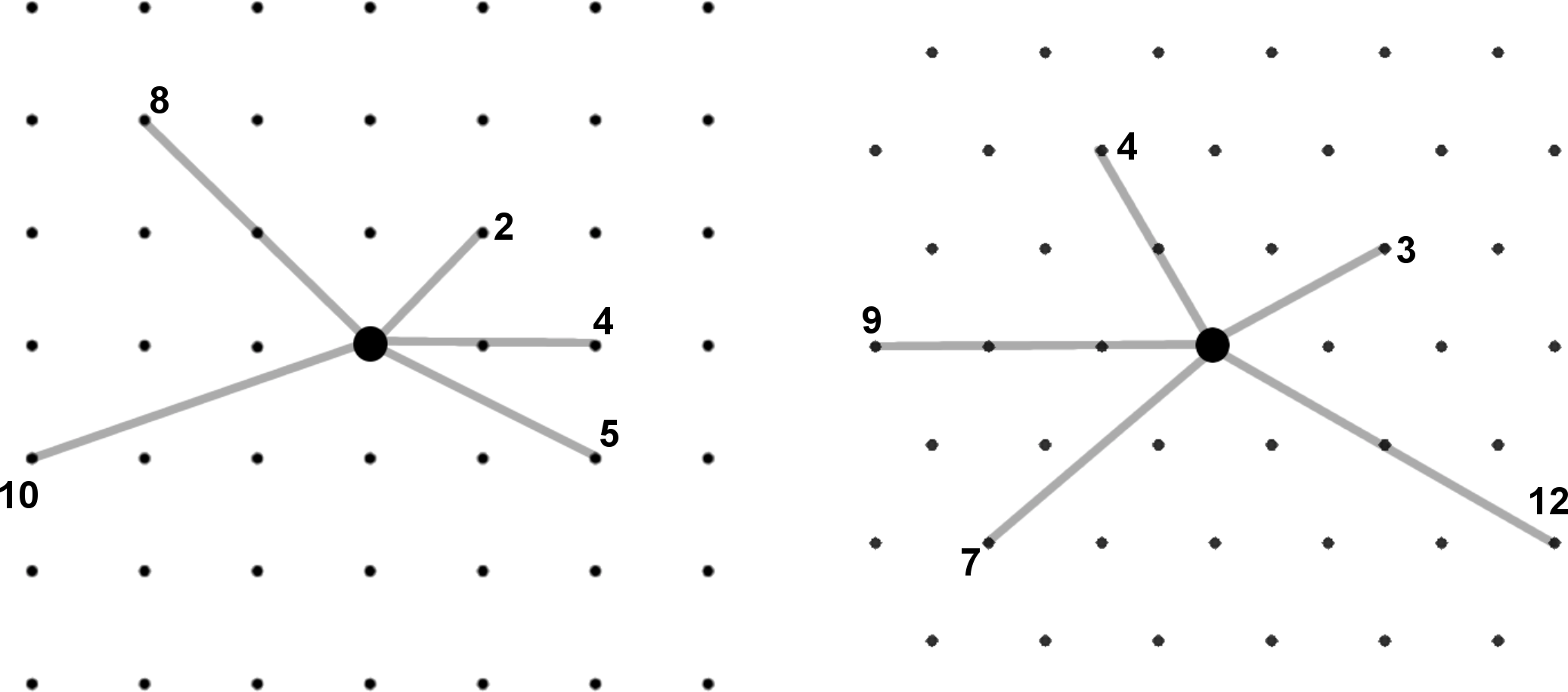Figure 4.4. Some unique spines, with norms Spines are not visually interesting, but they are conceptually important. Think of the spine as inherent potential in a family; the egg—before cell division starts. It is analogous to the “initiator”—the line segment that serves as the starting point for fractal iteration.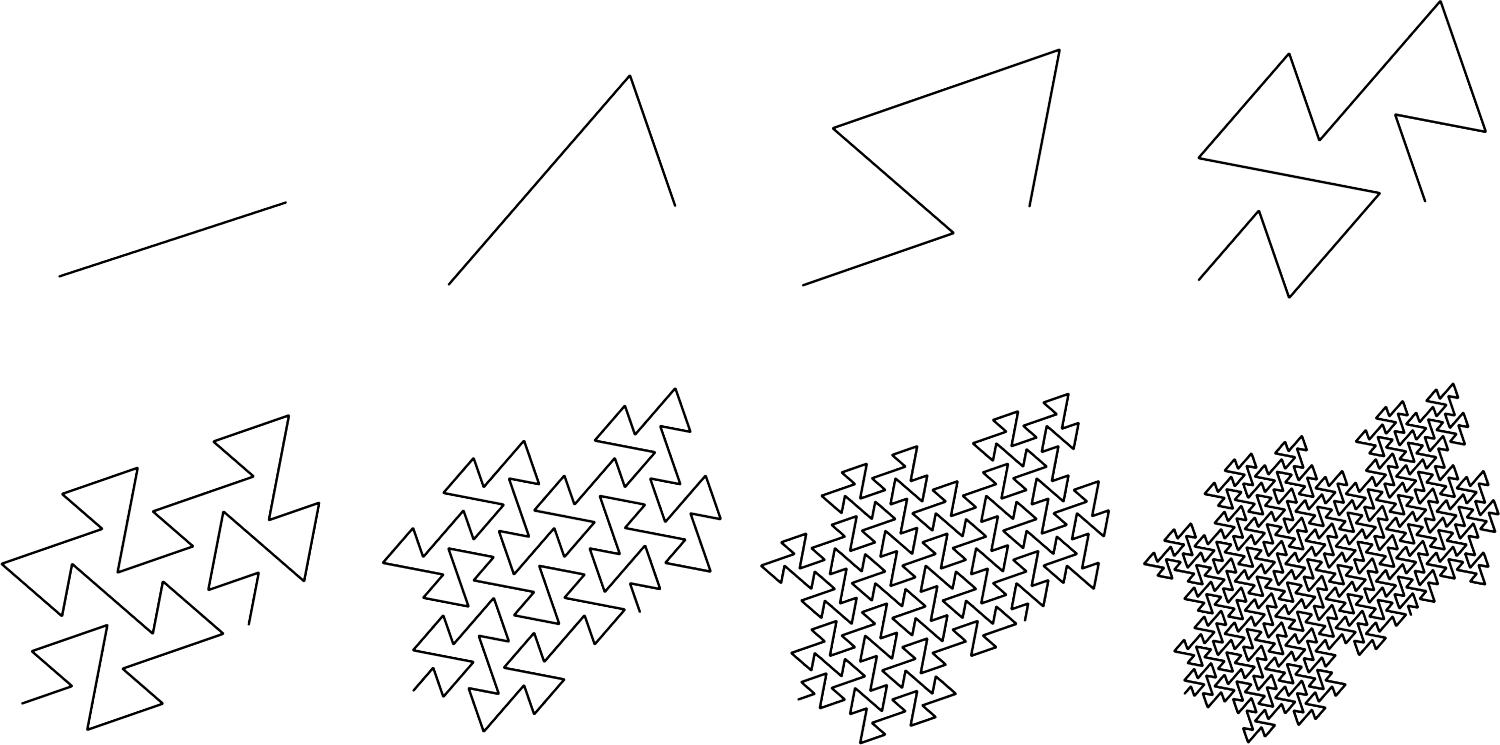Figure 4.5. Teragons 0-7 of a self-avoiding curve of the E(2,1) family Flesh If the spine is the initiator of the curve, then the flesh is the curve itself—the meandering line that fills a planar area. In a plane-filling fractal curve, flesh resolves to an infinitely-filled area. The way the curve fills the area varies greatly among curves. Mandelbrot called this the “sweep” of the curve . The pattern in this meandering curve can be seen more clearly in lower-order teragons, where the line is visible, as shown in Figure 4.6.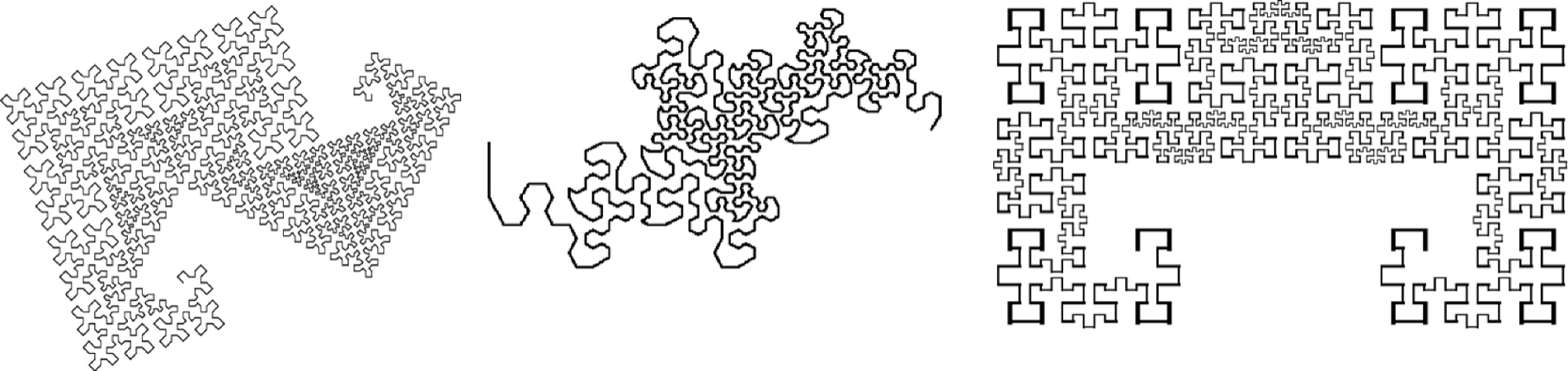Figure 4.6. Meandering curves constitute the flesh that fills the interior of the body shape As a plane-filling curve sweeps through its area of the plane, tracing out its history, it meets itself many times along the way—coming in close proximity to moments in its past journey. A two-dimensional polyrhythm is apparent in every curve’s sweep—as a distinct pattern (Figure 4.7).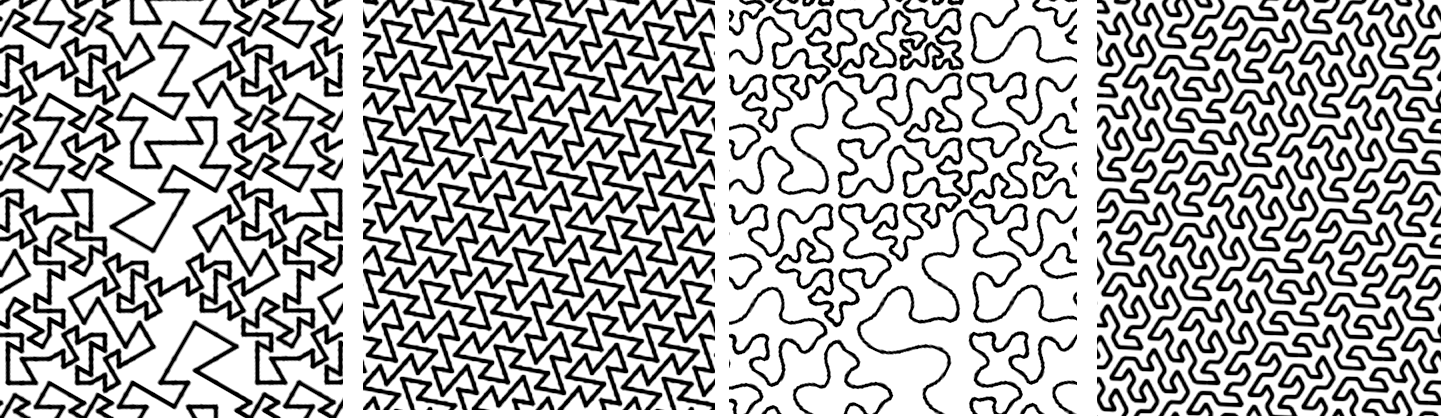Figure 4.7. Variations in flesh Power family curves with teragons having multiple-length segments (such as the Dragon of Eve, Mandelbrot’s Snowflake Sweep, and the curves shown in Figure 4.6) have variable amounts of local structure distributed within their flesh. This variability extends across more scales in the higher teragons. Describing the self-similar polyrhythm of the sweep that forms flesh is not easy, but there are some specific attributes that are easy to measure—in terms of how a curve can interact with itself during the sweep. There are four primary categories of self-interaction in a fractal curve: 1. self-avoiding 2. self-contacting (touching at a lattice point, but not crossing) 3. self-overlapping (sharing a common line segment) 4. self-crossing These are illustrated in Figure 4.8.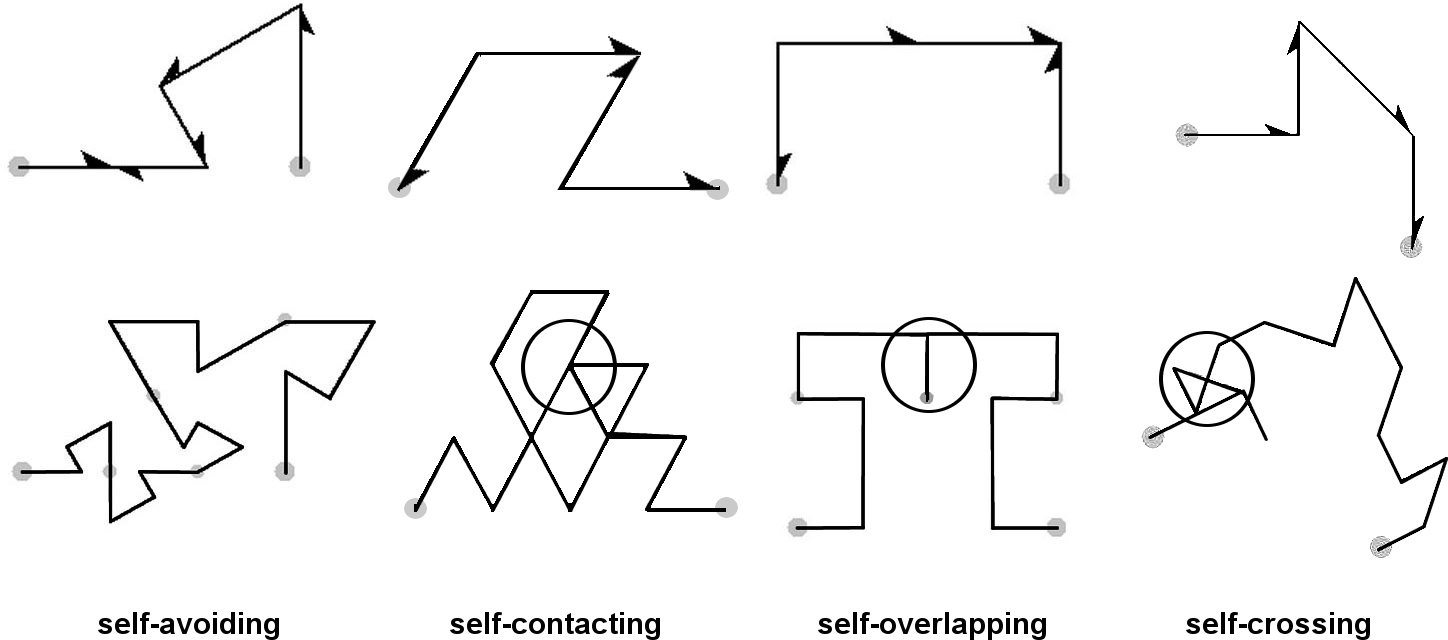Figure 4.8. Four ways a curve can interact with itself Self-Avoiding Curves Self-avoiding curves have a place of honor in the Kingdom of Curves—being the most clever and acrobatic of them all. A celebrated example is the Gosper curve , shown in Figure 4.9 (also called “flowsnake”).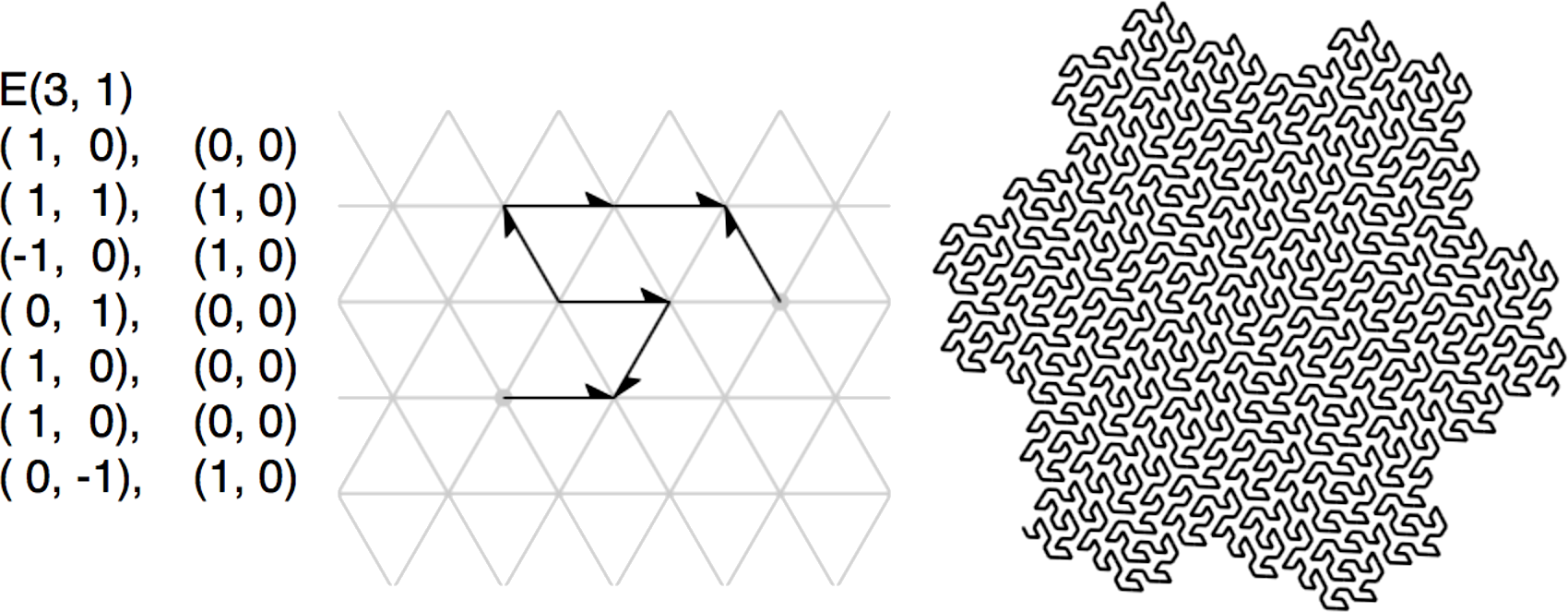Figure 4.9. The Gosper curve Many variations on the Gosper curve have been discovered by Fukuda, et al. , using a clever recursive replacement technique. One of these curves is illustrated in Figure 4.10, with visual overlay. This curve can be described as a member of the E(4,1) family, with norm 13.Figure 4.10. a variation of the Gosper curve by Fukuda et al. Self-avoiding curves often resemble the boundaries between two highly-convoluted domains. More self-avoiding curves will be introduced later in the book. Self-Contacting Curves Self-contacting curves touch themselves at points in the lattice, visiting common points in their sweeping histories. They come in many varieties in terms of how they self-contact. The most regular of these are the “lattice-fillers” or “grid-fillers” (Mandelbrot called these “chunks of lattice”). These curves touch themselves at every lattice point within the body. They can also be called “edge-covering”  since they traverse every edge of the grid once, within the body. (Figure 4.11).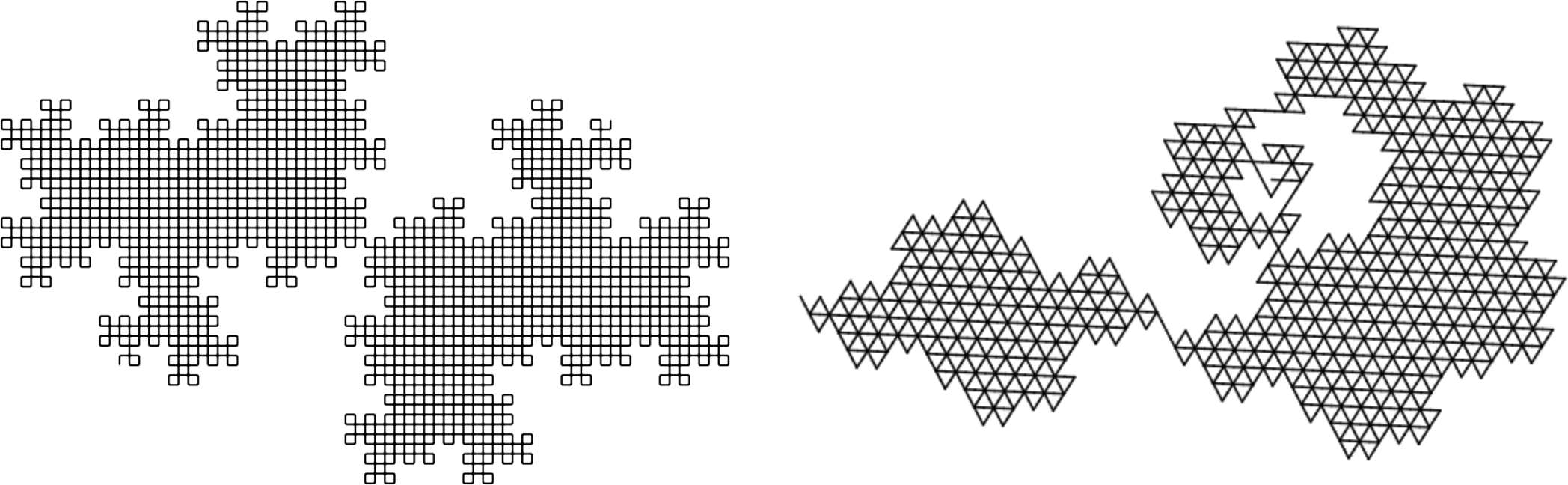Figure 4.11. The inverted 5-Dragon of the G(5,0) family and a curve of the E(3,1) family Splined Curves The angles in a lattice-filling curve can be smoothed-out and rounded (splined) to visually separate the contact-points, thus revealing the sweep of the curve. Otherwise it is impossible to see the direction of the sweep. Three examples of classic lattice-filling curves are the HH Dragon, the Terdragon, and the Original Peano curve, shown in Figure 4.12. The curves are splined to reveal their sweeps.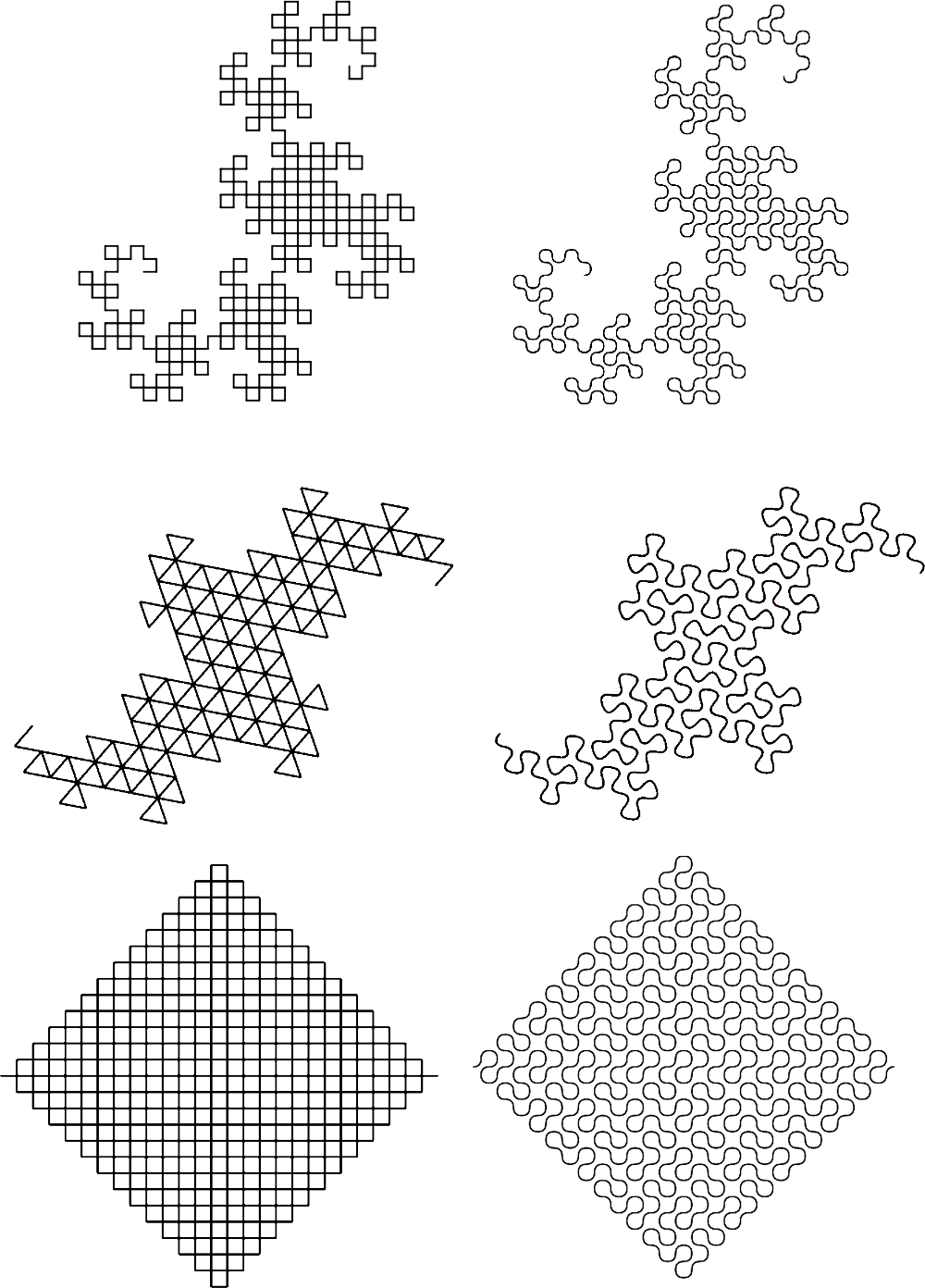Figure 4.12. Three classic lattice-filling curves (splined) Another example is shown in Figure 4.13: two different sweeps of the 7-Dragon. Again, the curves are splined to reveal their sweeps. (Since these curves do not require any transforms, they are not included in the genetic description.)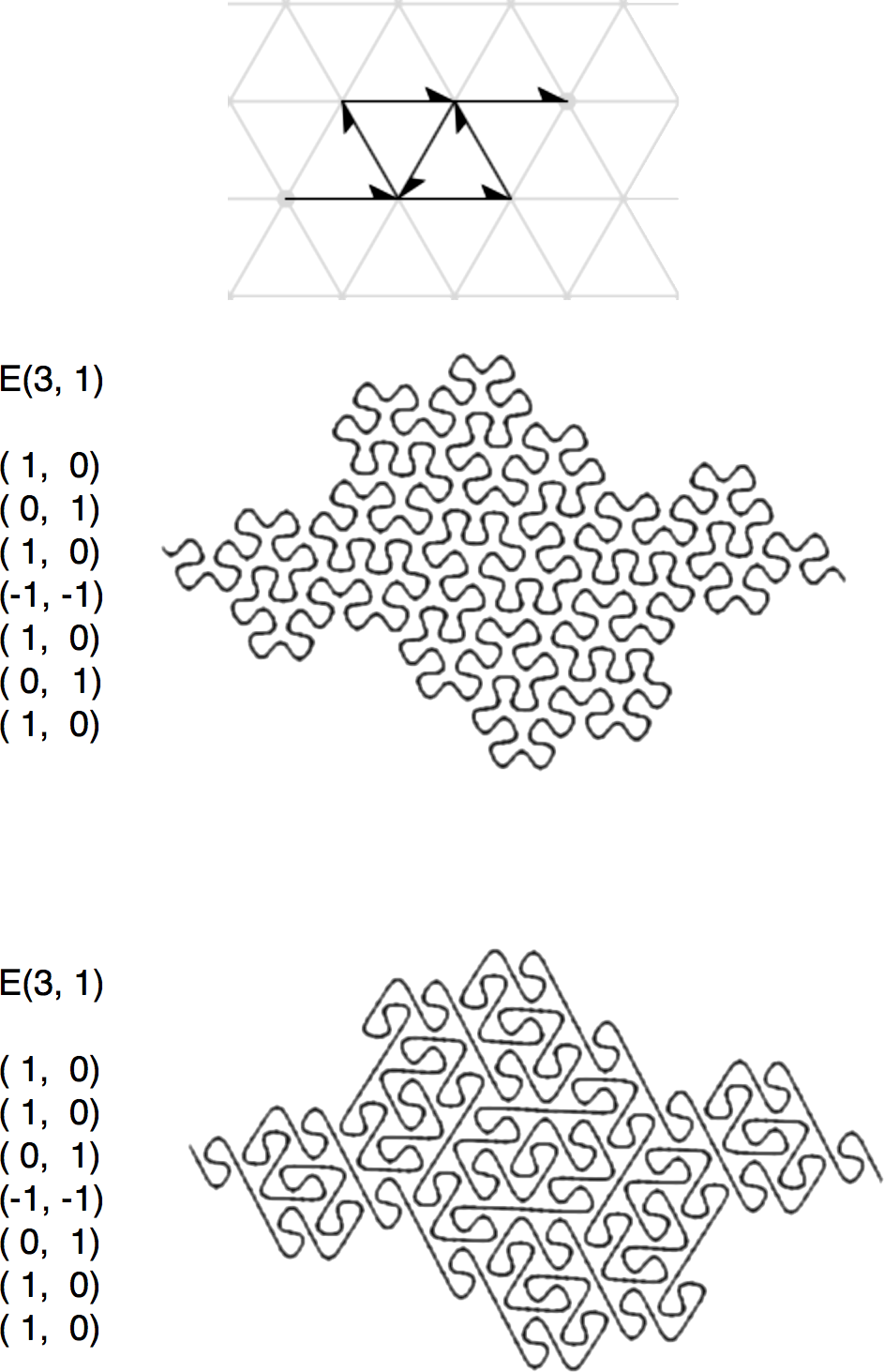Figure 4.13. Two variations (sweeps) of the 7-Dragon (splined) Unlike lattice-fillers, partially-self-contacting curves do not touch themselves at every lattice point within the body. Among the many examples are two subtly-different curves of the G(5,0) family (shown in Figure 4.14, with splined renderings). Their generators each have the same 5 integers of norm 5, but with different transforms. These two curves are related to a tiling described by Bandt et al. . At the bottom of the figure are 25 tiles that make up the shape. Like the two 7-Dragons above, these curves have identical body shapes, but their sweeps are different.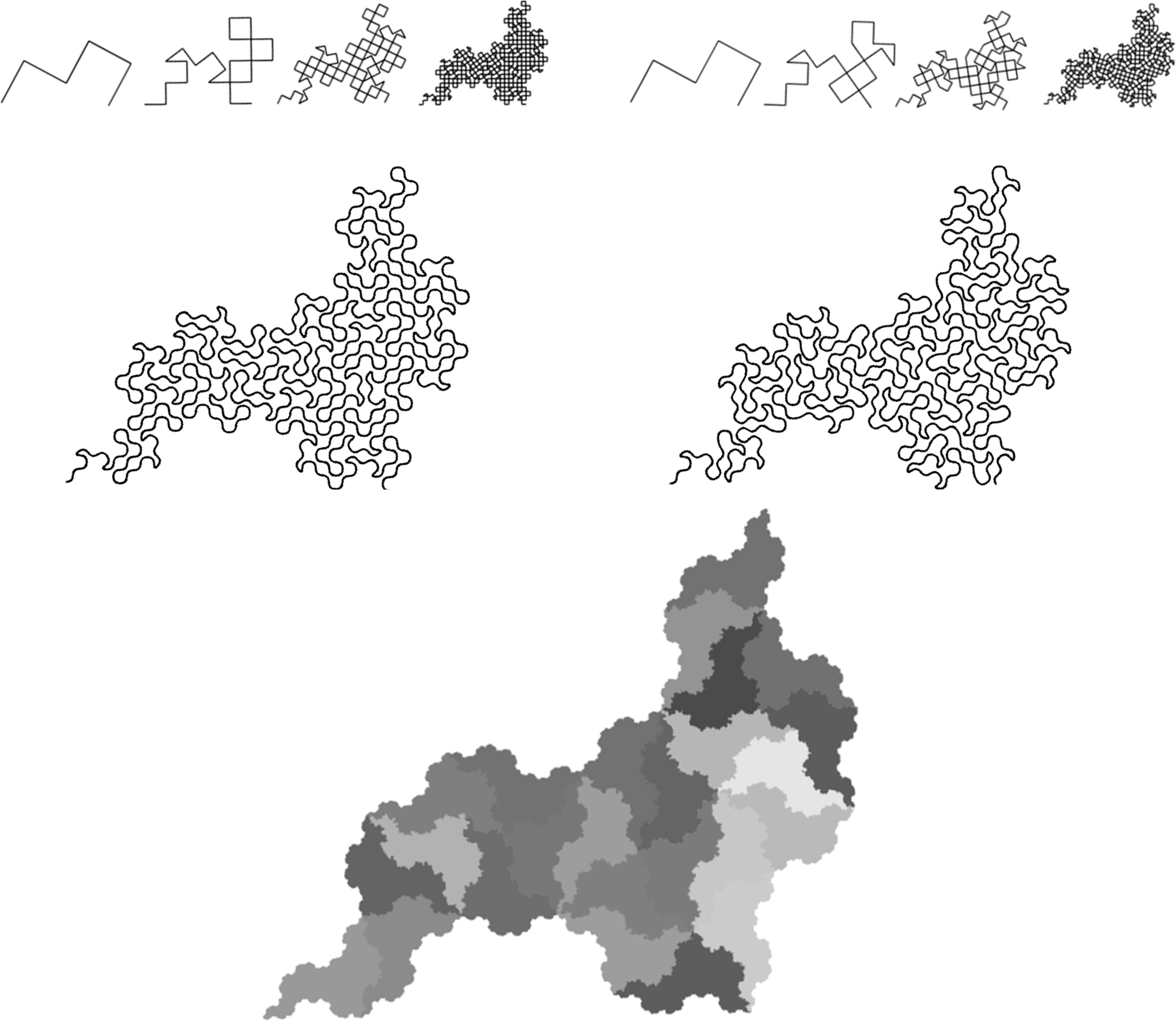Figure 4.14. Two curves from the G(5,0) family (splined) and a tiling by Bandt Self-Overlapping Curves If a generator has overlapping segments, it is guaranteed to produce teragons with overlapping segments. Some generators with no overlapping segments may still produce teragons with overlapping segments, which usually show up after a few iterations. Self-overlapping curves are usually not well-behaved; many of them have tremas and overlapping in their flesh as a result of uneven filling. But a few well-behaved self-overlapping curves are worth mentioning; they are part of the familiar celebrity set of fractal curve history. These curves include the Peano sweep (partially overlapping) and the G(2,0) version of the Cesàro Sweep (doubly-overlapping everywhere except the bottom edge), shown in Figure 4.15. (The Peano sweep is splined).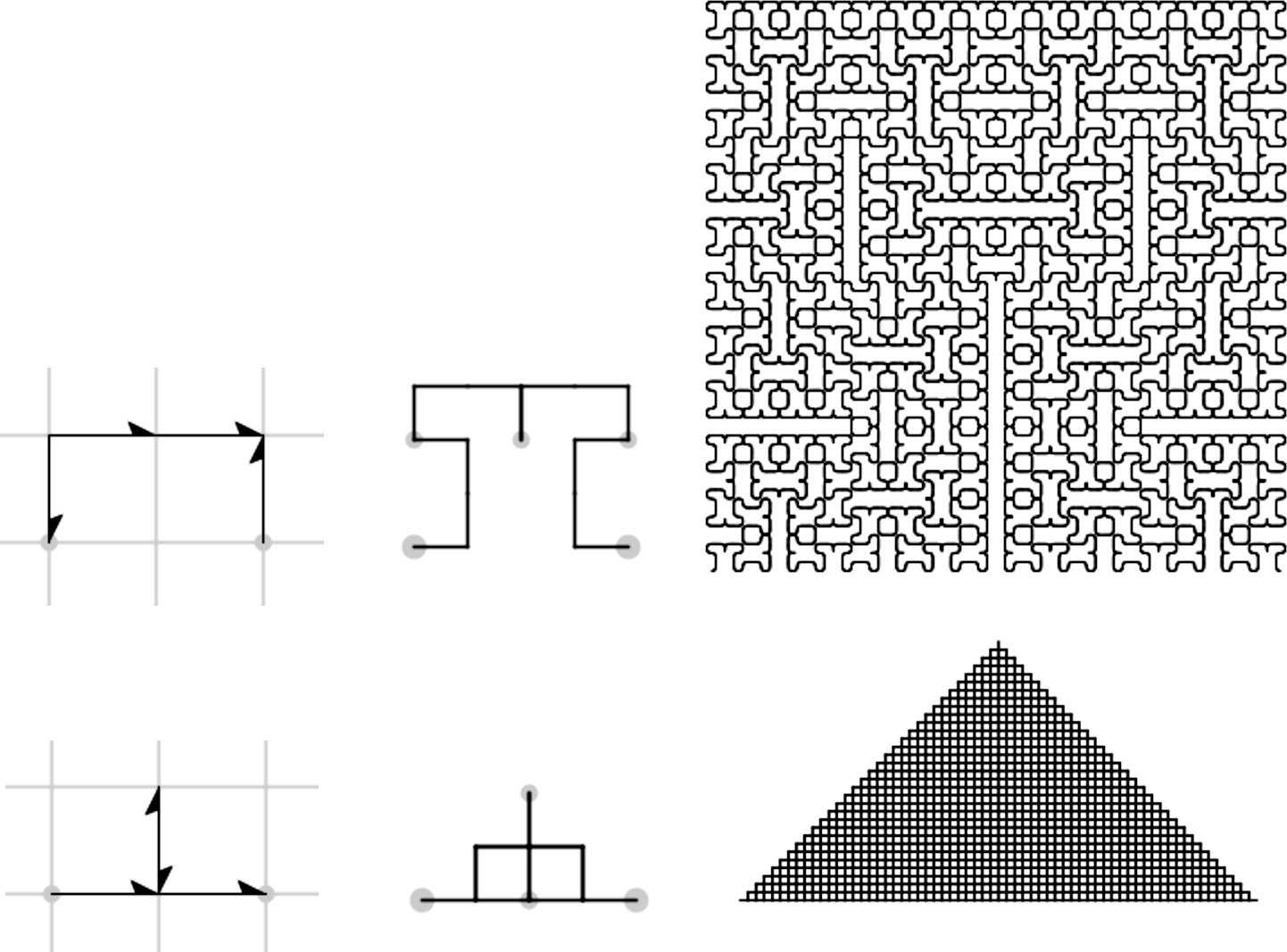Figure 4.15. Peano sweep and G(2,0) version of the Cesàro sweep are self-overlapping An interesting self-overlapping curve was identified by Adam Goucher , inspired by the pinwheel tiling . Its initiator is a right triangle with sides having lengths 1, 2, and √5 (top of Figure 4.16). It can be represented in the G(5,0) family by way of a reflection, a rotation, and a scaling by √5. The third and fourth integers of the generator are overlapping. At the bottom of figure 4.16 is a splined rendering.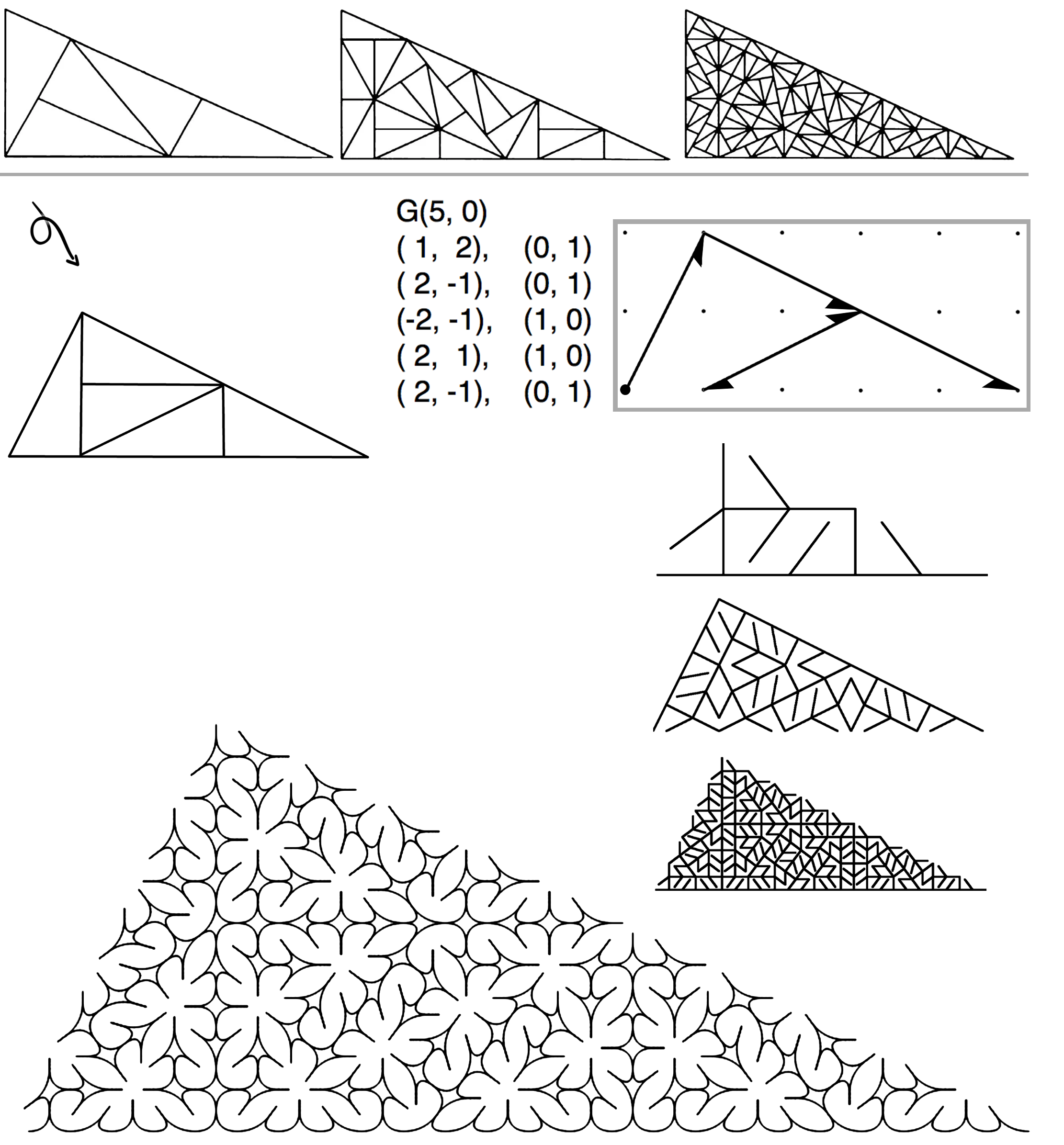Figure 4.16. The pinwheel tiling and a corresponding self-overlapping curve Several self-overlapping curves on right triangles have been identified, including one related to a node-replacement curve by Teachout  (top of Figure 4.17). Below that is the associated edge-replacement curve, which is splined to reveal its sweep.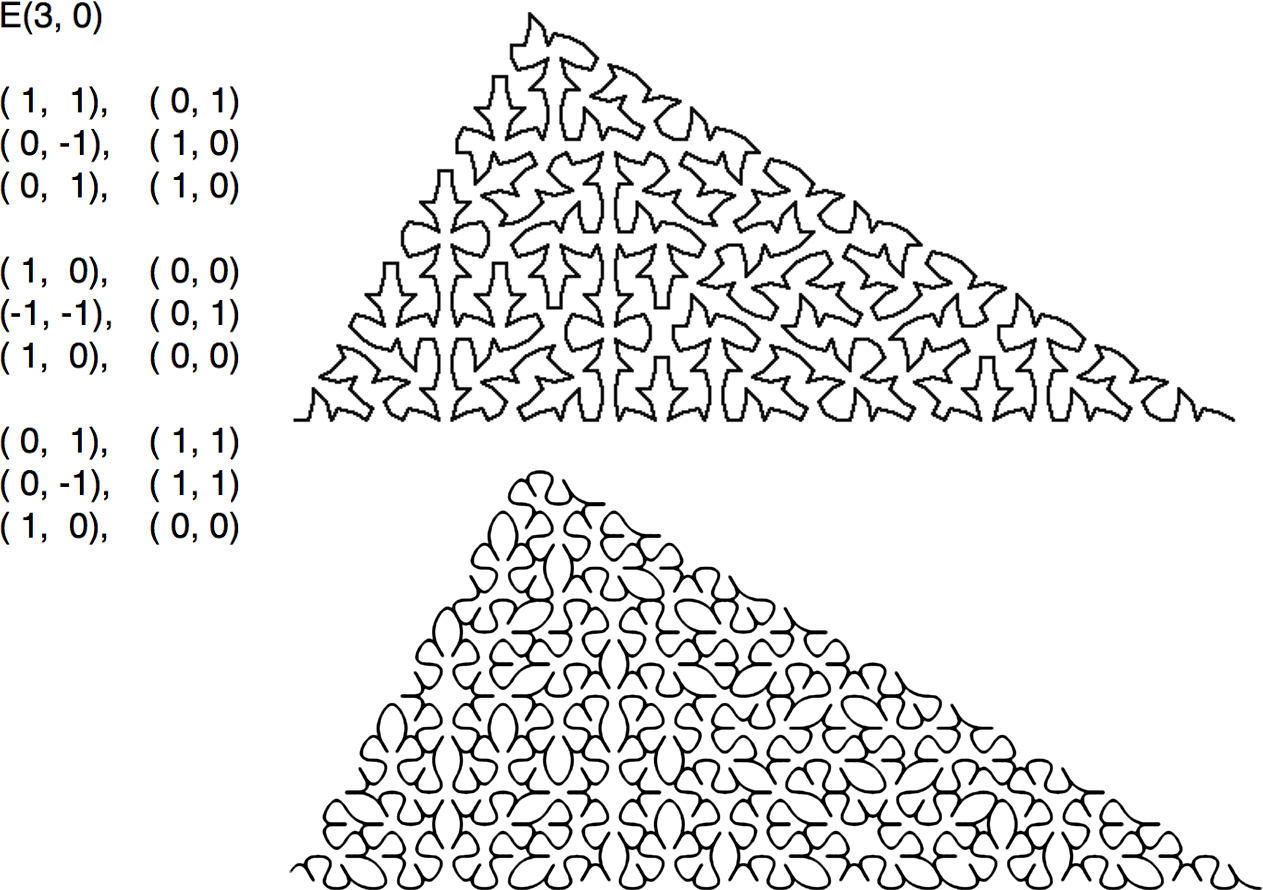Figure 4.17. A curve by Teachout (top) and a related edge-replacement curve (splined) Self-Crossing Curves When considered as an unordered collection of line segments that happen to share vertices, self-crossing curves are identical to self-contacting curves. The difference lies in their sweeps, where ordering is important. Self-crossing curves can be clever in their Celtic knot-like sweeps. Figure 4.18 shows a self-crossing variation of the 7-Dragon shown at the top of Figure 4.13. In this drawing, the curve is splined so that the tangled rhythm of its sweep can be appreciated.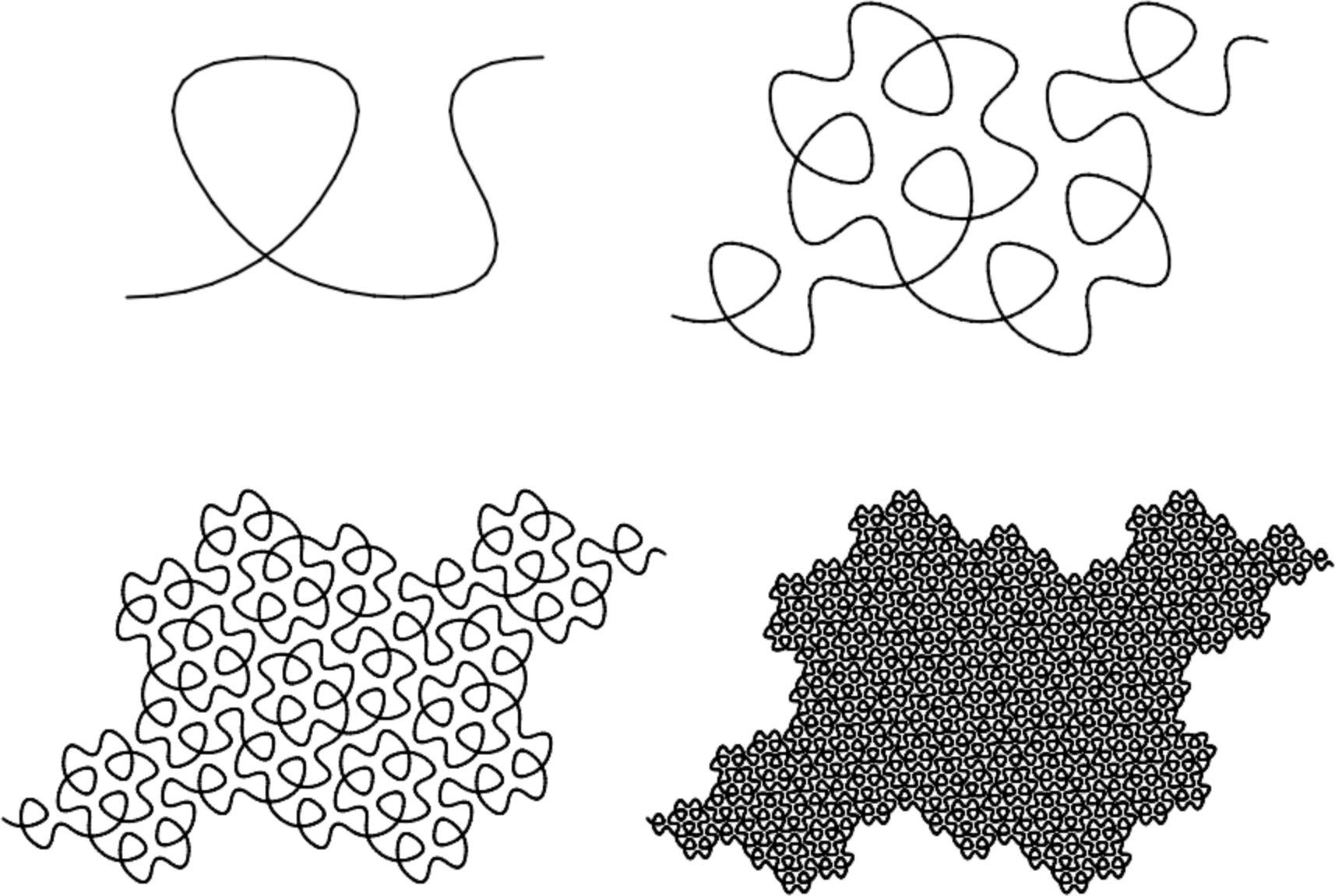Figure 4.18. A self-crossing variation of the 7-Dragon (splined) Skin Skin is a higher-order emergent attribute of plane-filling curves. It comes into form through the process of iterating teragons—filling ever-finer chunks of the plane with meandering flesh. A boundary is revealed as the process continues, and it becomes crisp to the eye when the teragon order reaches the visual threshold of detail. Other than this visual, impressionistic description, it is difficult to define skin, since it is a chaotic subset of flesh: the bits that lie at the edge of the body shape—which itself is emergent. Perhaps because of this fragmented nature, skins have peculiar properties; most of them are fractal curves in themselves—and they are intimately related to the recursive nature of the flesh within the body shape, through it may not be straightforward to describe this relationship. Conjecture: the fractal profile of skin is unique to each family set. If this is proven to be true, what might the reason be? A single family can have multiple kinds of skin. For example, the E(3,1) family with norm 7 has several curves with “Gosper skin” (having the same jagged profile as the Gosper curve), but also other skins that are completely different, and highly convoluted (Figure 4.19).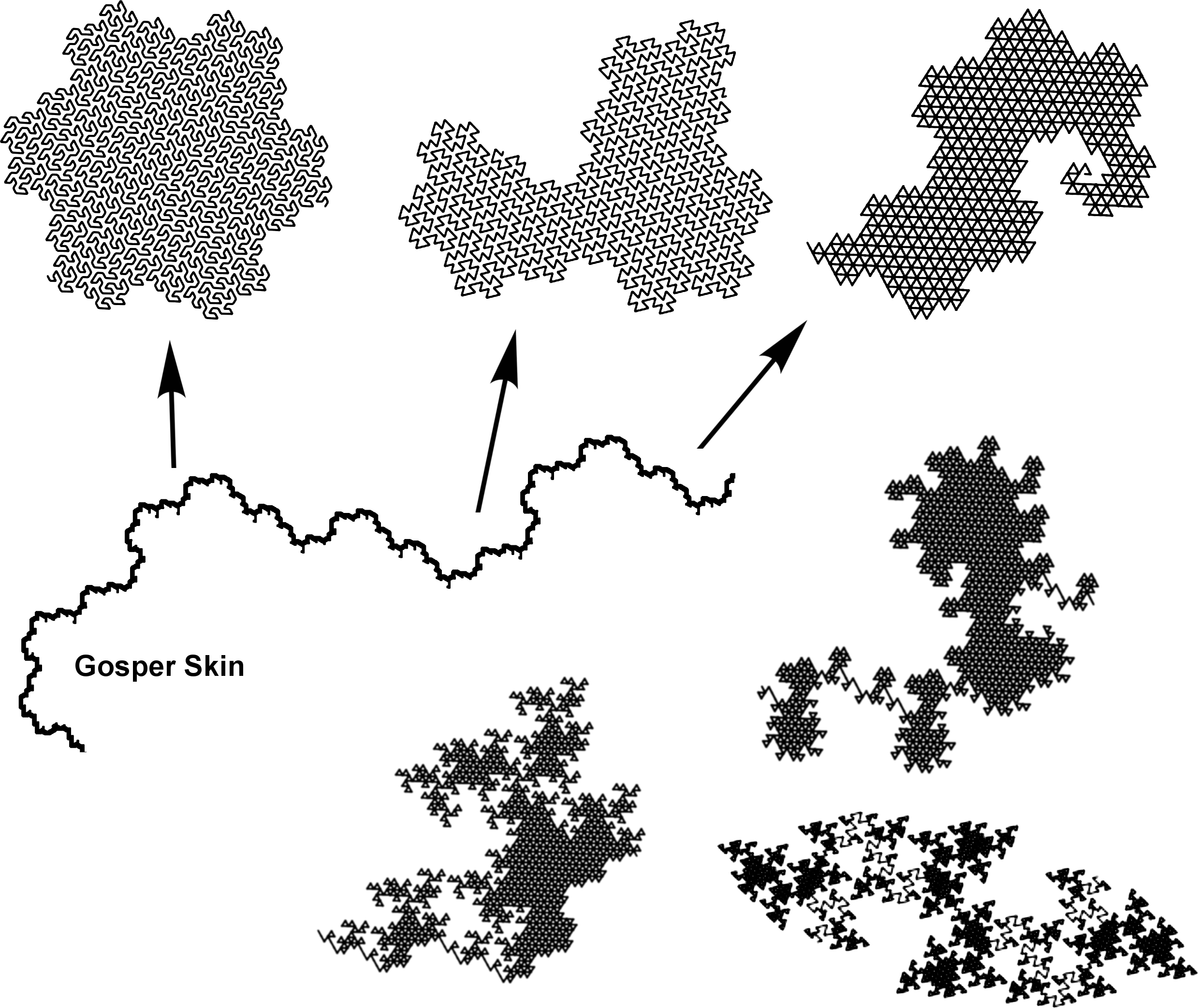Figure 4.19. Gosper skin can be found in several curves of the E(3,1) family “Skin” denotes the fractal quality of a curve’s boundary independent of its gross morphology: the skin’s unique signature profile remains intact, no matter what scale of magnification is used to view it. Gross morphology, on the other hand, is a property that holds for one magnification only: the lowest. For example, figure 4.20 shows select sections of the skin of the HH Dragon—described by Chang and Zhang .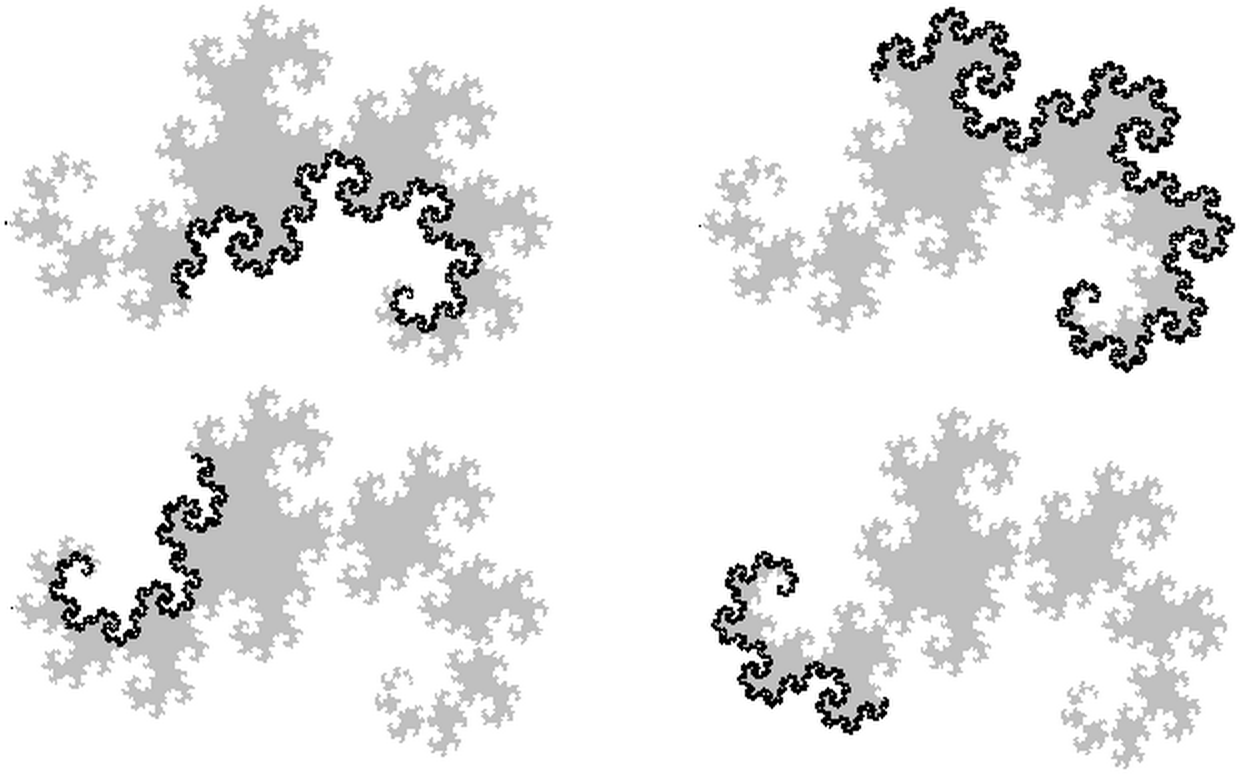Figure 4.20. HH Dragon skin described by Chang and Zhang The fractal dimension of skin The fractal dimension of HH Dragon skin is 1.52362. The fractal dimension of Gosper skin is 1.12915. The skin of the Terdragon (Figure 4.21) has a fractal dimension of 1.26186 (which happens to be the same as the Koch curve).Figure 4.21. Terdragon skin The fractal dimension of skin varies among curves. Consider the three curves shown in Figure 4.22.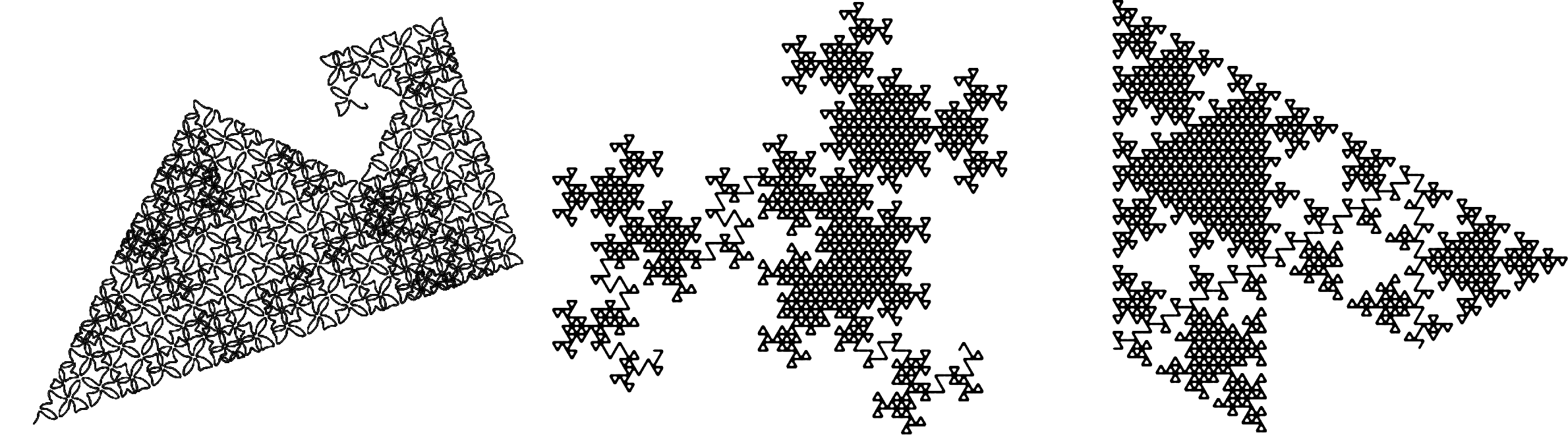Figure 4.22. Examples of various skins with different fractal dimensions The curve at left has a skin with dimension 1: any magnification of the boundary appears as a straight edge—with the exception of a set of convex and concave corners, most of which appear near the hook at the top. The skin of the curve in the center of the figure has a high dimension, and at the limit it has an infinity of self-contacting points that pinch-off the body to form an infinity of kissing topological disks. And the curve at right…? Self-Avoiding Skin vs. Gasket Skin The curve at the right of Figure 4.22 has a skin whose dimension is ambiguous: the boundary contacts itself so much that it is indistinguishable from its own flesh, creating myriad cavities (tremas). It is similar to a gasket (a fractal with dimension < 2 that has an infinite number of tremas everywhere in its flesh). But can it be called a gasket? It has solidly-filled areas—whose interiors remain solid (locally plane-filling) at arbitrary magnification. Perhaps it could be described as a plane-filling curve whose skin is a gasket. Similar to the way a curve’s flesh can interact with itself in many ways, a skin with high dimension may exhibit acrobatic self-contacting yoga. When a curve fills an area that is topologically equal to a disk, then it is a straightforward exercise to identify skin from flesh. It is a self-avoiding skin, making a clear delineation between inside-body and outside-body. The curve at the right of Figure 4.22 deviates from such topological clarity because its sweep leaves tremas, making it impossible to distinguish its skin from its hole-ridden flesh. Interestingly, a higher-level emergent “boundary” is formed—in this case, it clearly appears straight—forming a broken-triangle-shaped polygon at the macro scale. Rather than try to identify skin from flesh in this class of curve, let’s just set it aside as a topological outlier—it is essentially an infinite set of kissing triangles (Figure 4.23).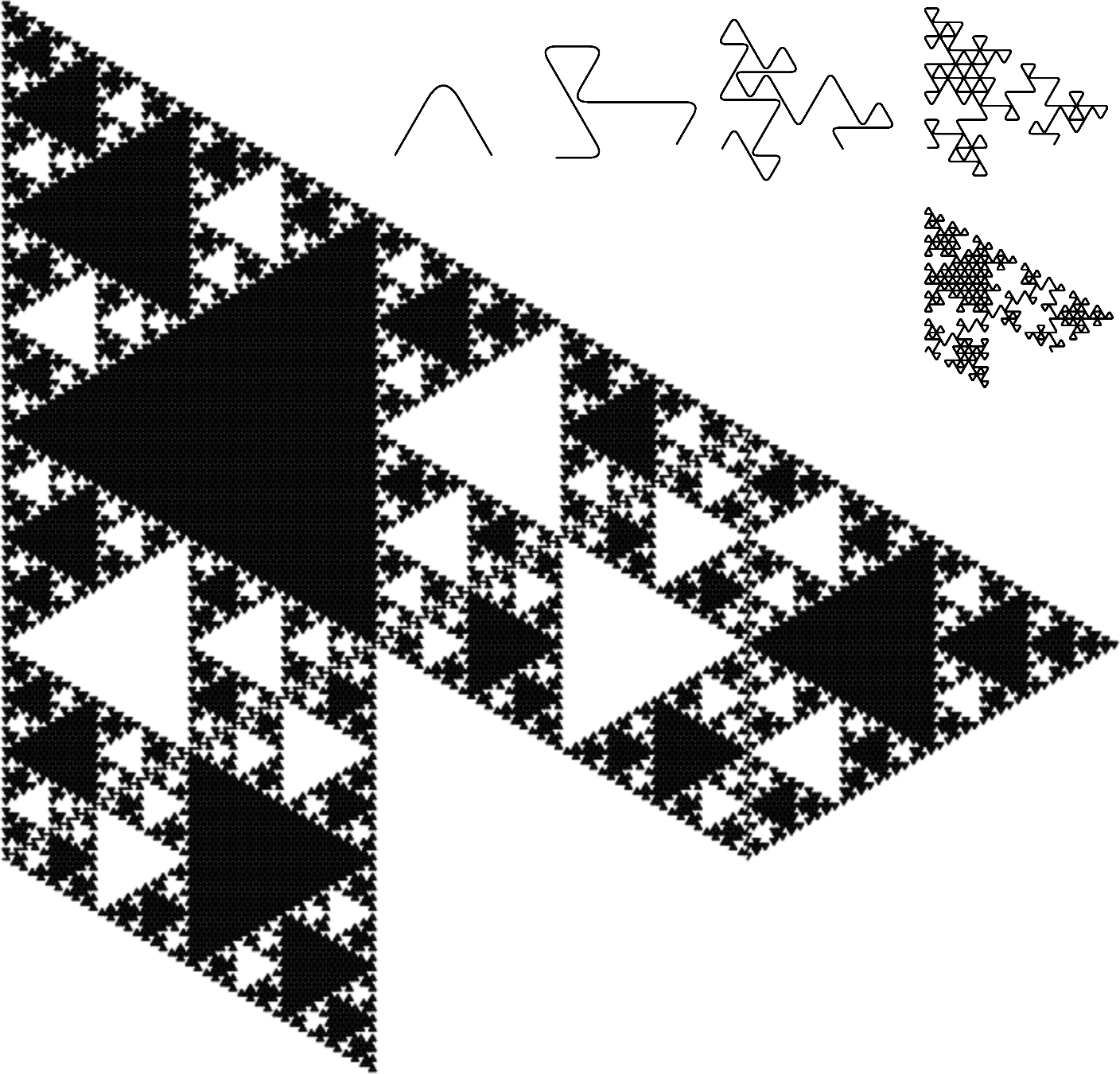Figure 4.23. A curve of the E(2,0) family This curve is reminiscent of a fractal described by Mandelbrot  (Figure 4.24), as well as the curve shown in Figure 3.11. These gasket-like pseudo-space-filling curves, and some variations of the Sierpinski triangle, share a common feature: “high lacunarity”—a term referring to fractals having tight clumps and large tremas.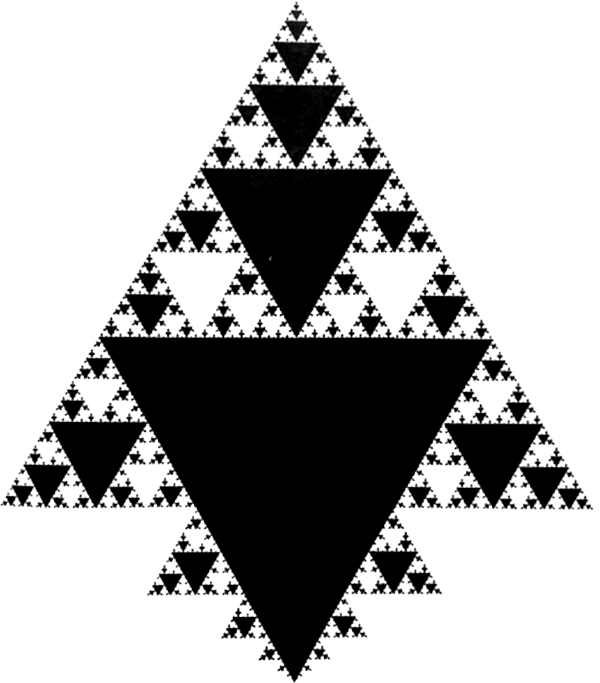Figure 4.24. A high-lacunarity fractal introduced by Mandelbrot Area Fractal generators are often described with the assumption that the distance between the endpoints is 1, as with the initiator Mandelbrot and others have used: the unit interval [0,1]. In contrast, the taxonomy described in this book represents the initiator as a 2D vector equal to the family integer. This is illustrated in Figure 4.25, using the Gosper curve as an example. Unlike Mandelbrot’s initiator, the distance between endpoints is √7 and each segment has length 1.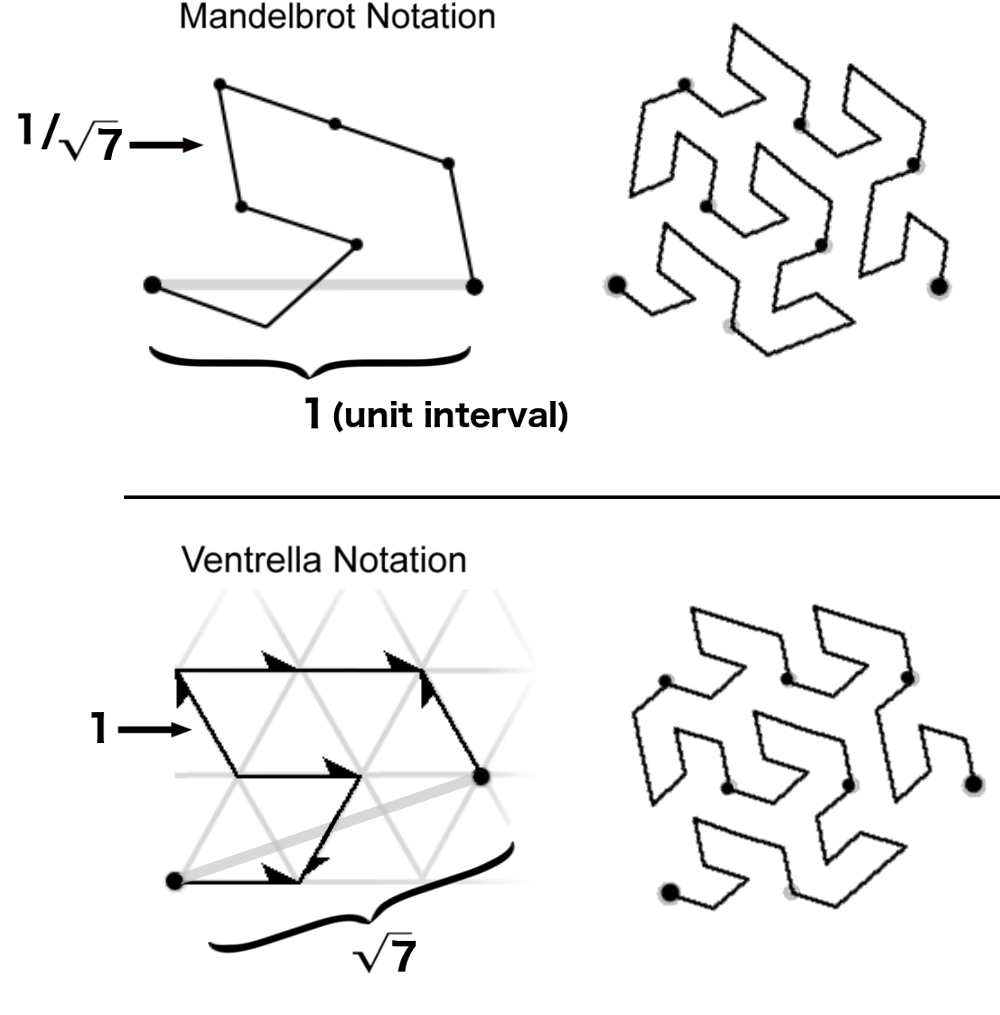Figure 4.25. Using the family integer as the initiator instead of the unit interval Family integers have varying norms. Assuming all teragons are normalized to occupy the family space, their areas vary as a function of family norm. This means that curves of higher family norms are larger. The smallest two curves are the HH Dragon and the Pólya curve of the G(1,1) family, each having an area of 1. Based on experimentation, a few observations have been made in terms of area: if it can be verified that a curve is non-overlapping, then its generator can be used to determine its area. The area of a curve in the Gaussian domain appears to be confined to the range between f/2 and f, where f is the family norm. In the Eisenstein domain, the area is confined to the range between f * √3/2 and f * √3. In the case of self-overlapping curves, the area appears to be smaller, as in the G(2,0) version of the Cesàro sweep, shown in Figure 4.26.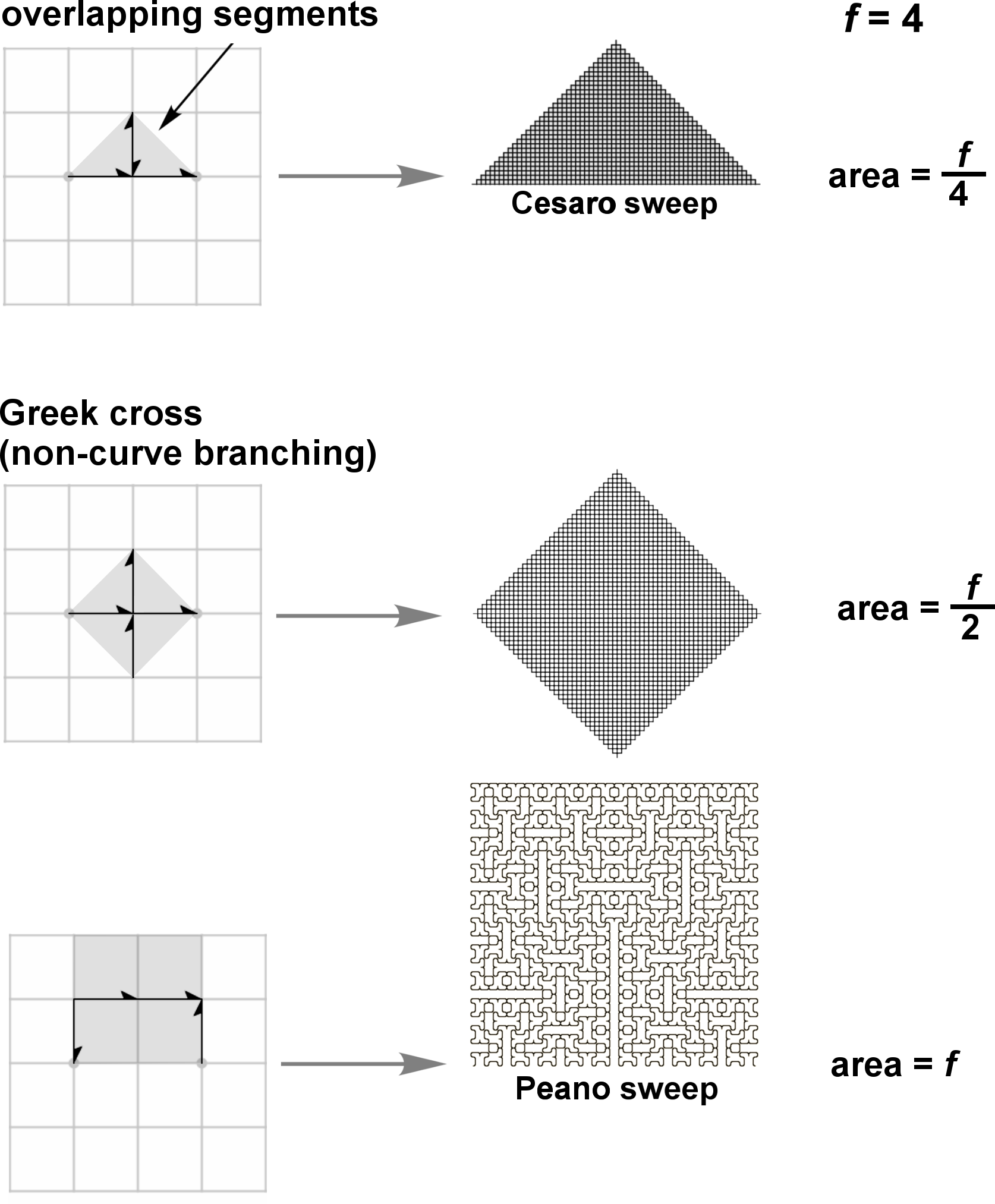Figure 4.26. Areas of the G(2,0) Cesàro sweep, Greek cross, and Peano sweep In the Gaussian domain, lattice-filling curves visit every lattice point exactly twice. In the Eisenstein domain, exactly thrice. Self-avoiding curves visit every lattice point once, and have the largest areas relative to other curves of the same family. (Conjecture: a plane-filling curve cannot have an area that is larger than its family norm). Tiling For curves whose integers all have the same norm (segments of the same length), tiling can be used to visualize the area—using identical tiles (monohedral tiling) that are placed on each line segment of the curve. A few of the basic types of monotiles that can be used are shown in Figure 4.27.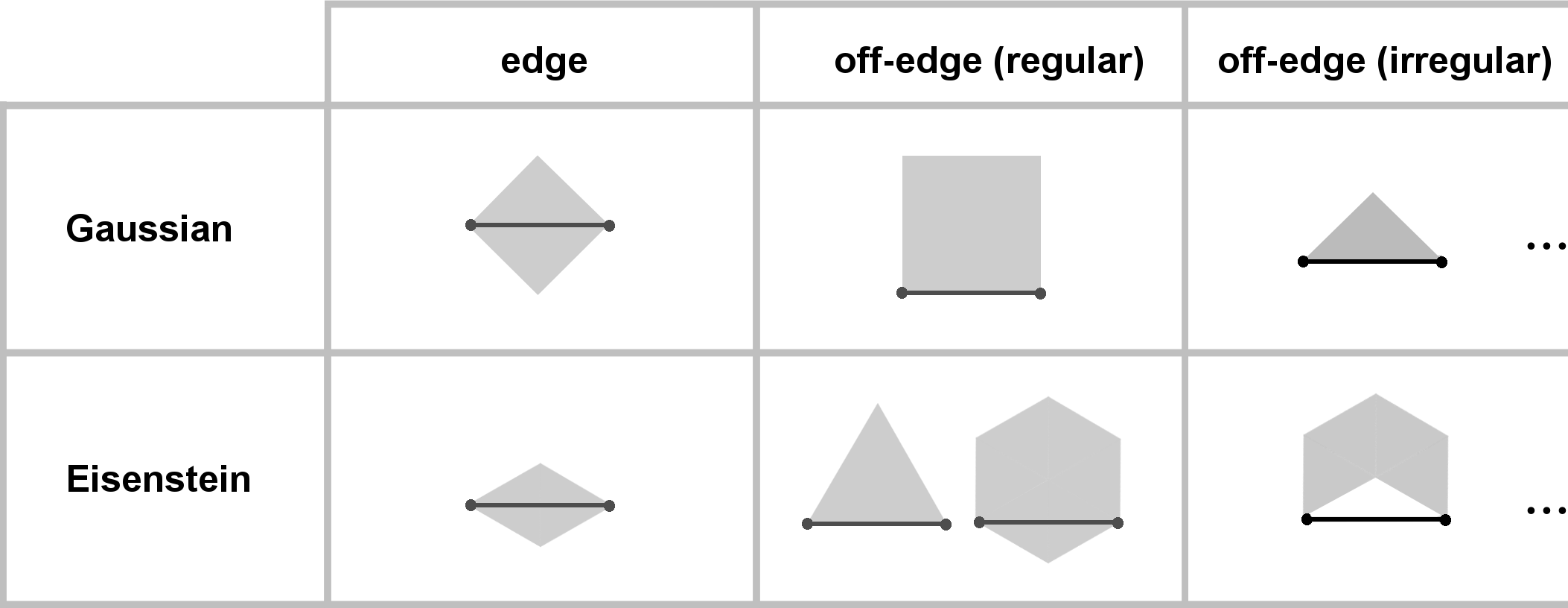Figure 4.27. Monotiles used for curves with integers having the same norm Lattice-filling curves can be tiled with edge-tiles, which are symmetric about the line segment, as shown in Figure 4.28.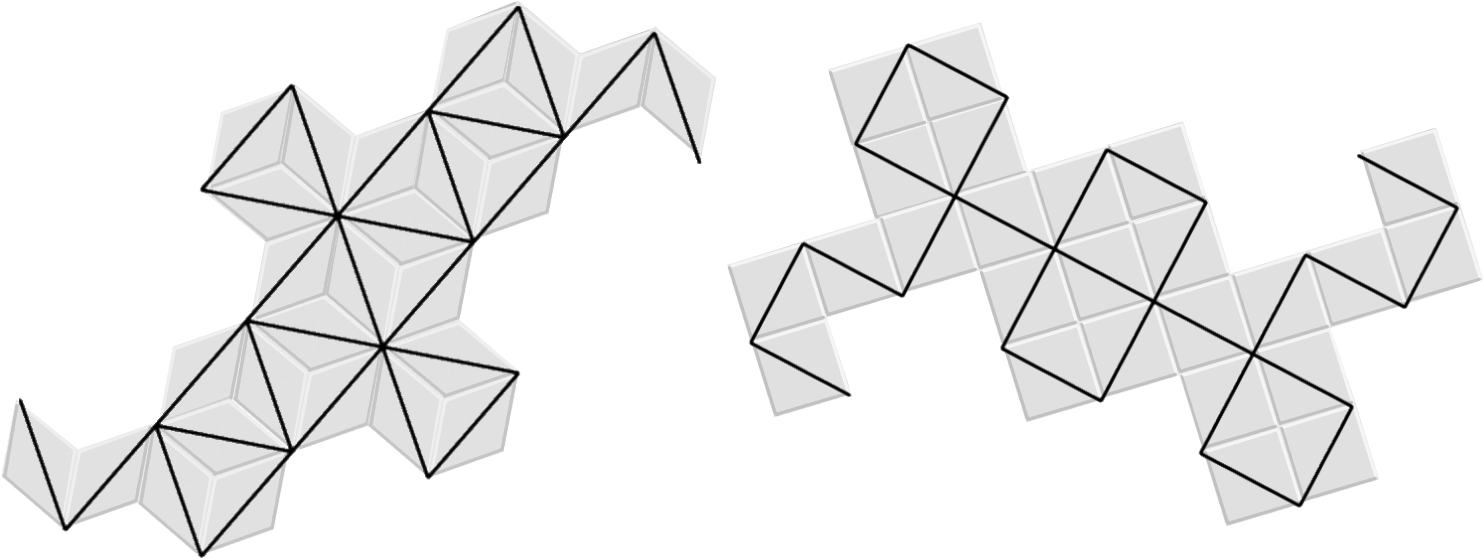Figure 4.28. Tilings of lattice-filling curves (Terdragon and 5-Dragon) Self-avoiding curves correspond to off-edge tiles. An example is the use of the regular hexagonal tile for tiling the Gosper curve, shown in Figure 4.29. (The entire shape of the Gosper curve is called the “Gosper Island”).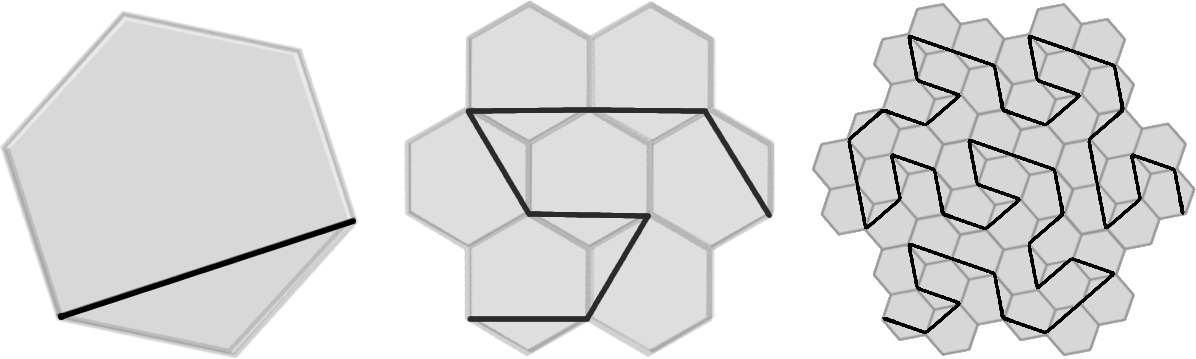Figure 4.29. Hexagonal tiling in the Gosper curve In the hexagon at the left of Figure 4.29, the length of the inscribed line segment is √7. If this were a segment of unit length, then the area of the hexagon would be √3/2. Multiplying that by √7 gives ~2.291287: the area of the Gosper curve. Figure 4.30 shows the four smallest squares in the Gaussian domain whose vertices lie on points in the lattice. Their areas are 1, 2, 4, and 5. The lengths of their edges are √1, √2, √4, and √5. Each square (other than the smallest) is accompanied by a plane-filling curve whose family integer traverses a square edge.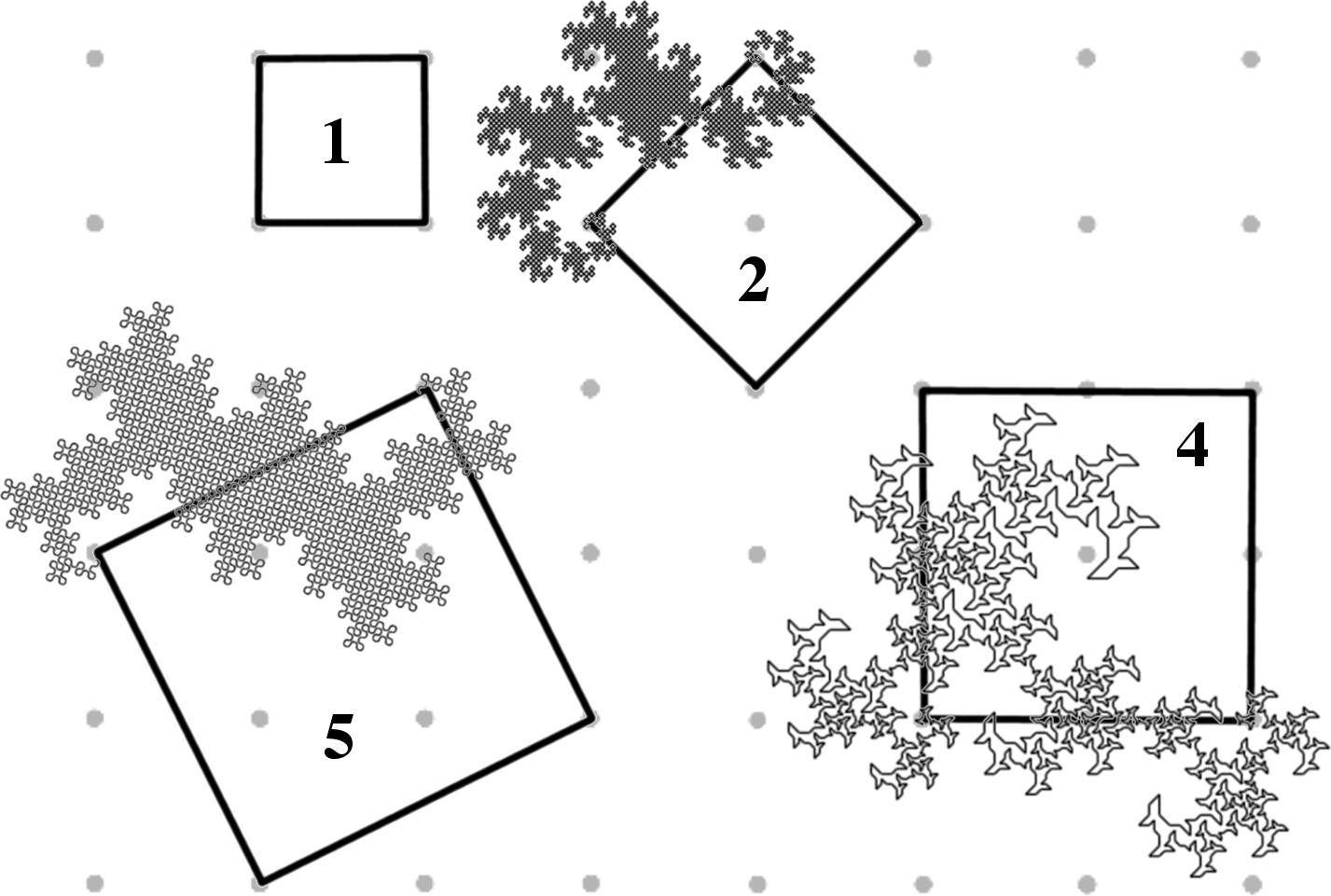Figure 4.30. Squares in the Gaussian domain with accompanying curves The HH Dragon (norm 2) and the 5-Dragon (norm 5), which are both lattice-fillers, have areas equal to one-half the area of their associated squares. Because the Dragon of Eve (norm 4) is a self-avoider, the assumption is that its area is equal to that of the square. However, verifying its area is a bit tricky…for the following reason: the Dragon of Eve has integers of varying norms, and so it does not lend itself to regular tiling. Figure 4.31. shows a progression of square tilings applied to the Dragon of Eve to visualize the area. The sum of the areas of the squares equals the family norm, which is 4. But tremas appear in teragon 3, and in teragon 4, there are tiles that overlap. More gaps and overlaps accumulate with each teragon. So, in this case, monotiling appears to be an unreliable means for determining the area.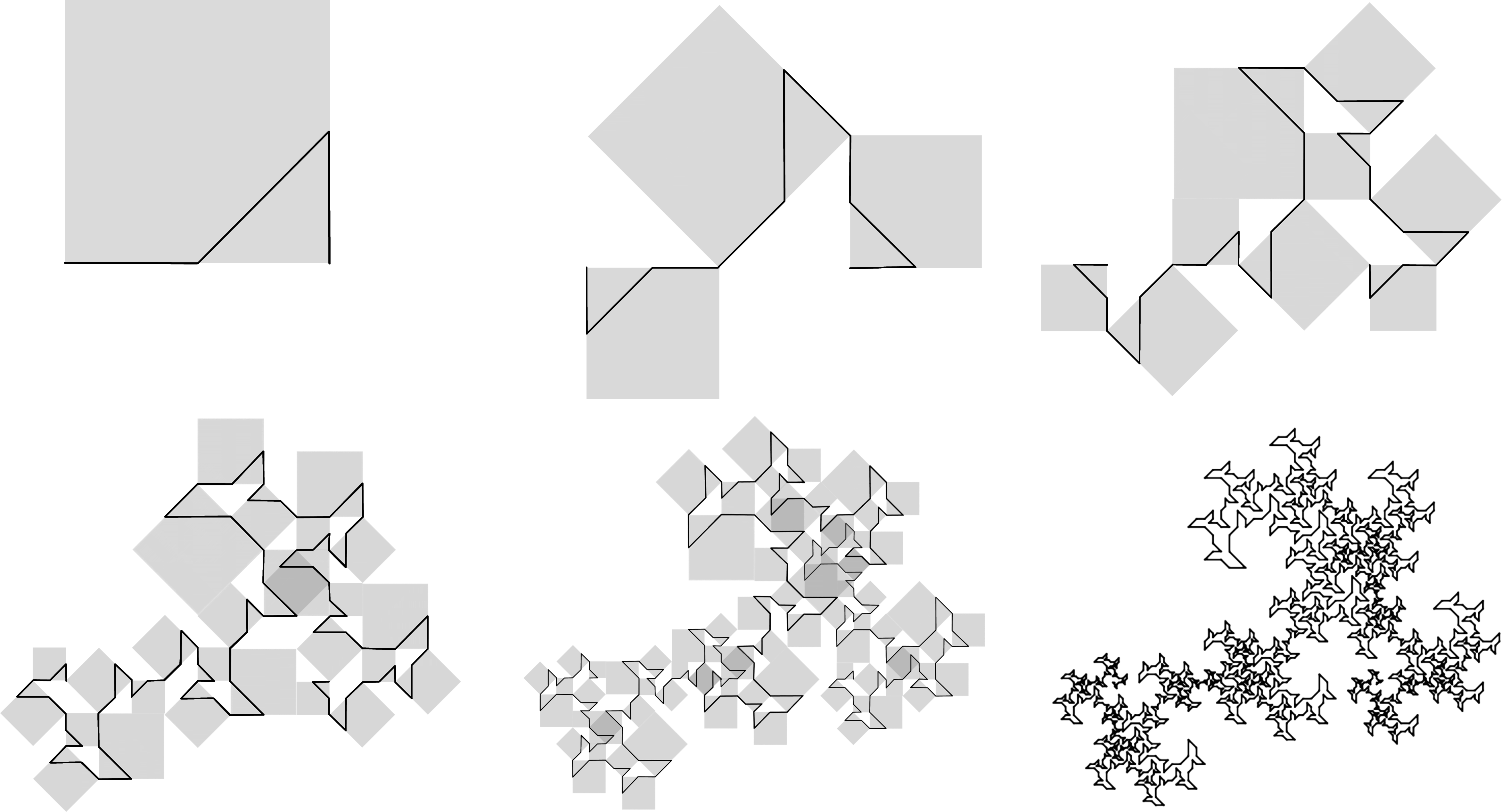Figure 4.31. Failed attempt at tiling the Dragon of Eve to calculate area This leaves us with a question: Is there any way to tile the Dragon of Eve with anything other than small copies of the curve itself? If not, what is the best way to calculate its area? The “broken hex” monohedral tile shown in Figure 4.27 can be used to tile the “fractal chair,” described by Bandt . This is shown in Figure 4.32, accompanied by teragons 0-7 of its associated curve in the E(2,1) family (splined).Figure 4.32. The fractal chair and its monohedral tiling, with teragons 0-7 (splined) Curvature Curvature refers to the amount of angular turning in a curve’s path from start to finish. It is defined here as the average angle between consecutive segments in the curve, where “angle” is a real value ranging from 0.0 to 1.0, as illustrated in Figure 4.33.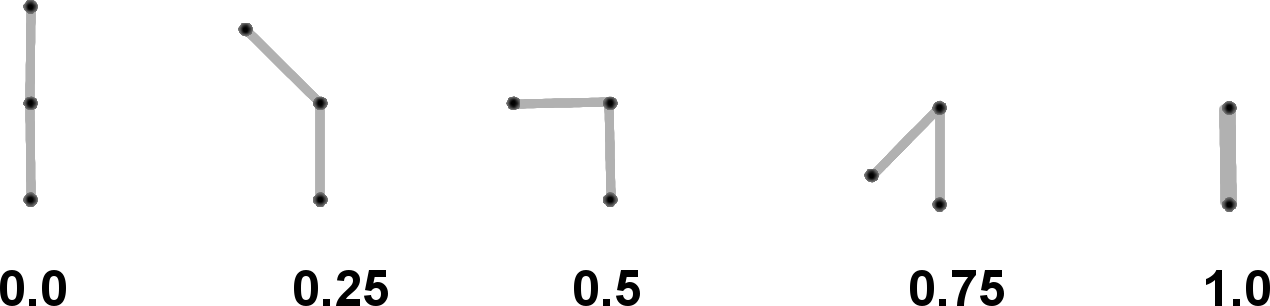Figure 4.33. Values for curvature between connected segments in a curve In the context of a single family, a larger area appears to be correlated with lower curvature. Consider the generators of two curves of the G(2,2) family, shown in Figure 4.34. The top curve is a lattice-filler, and the bottom curve is a self-avoider. Both curves have the same spine of length √8, shown at left.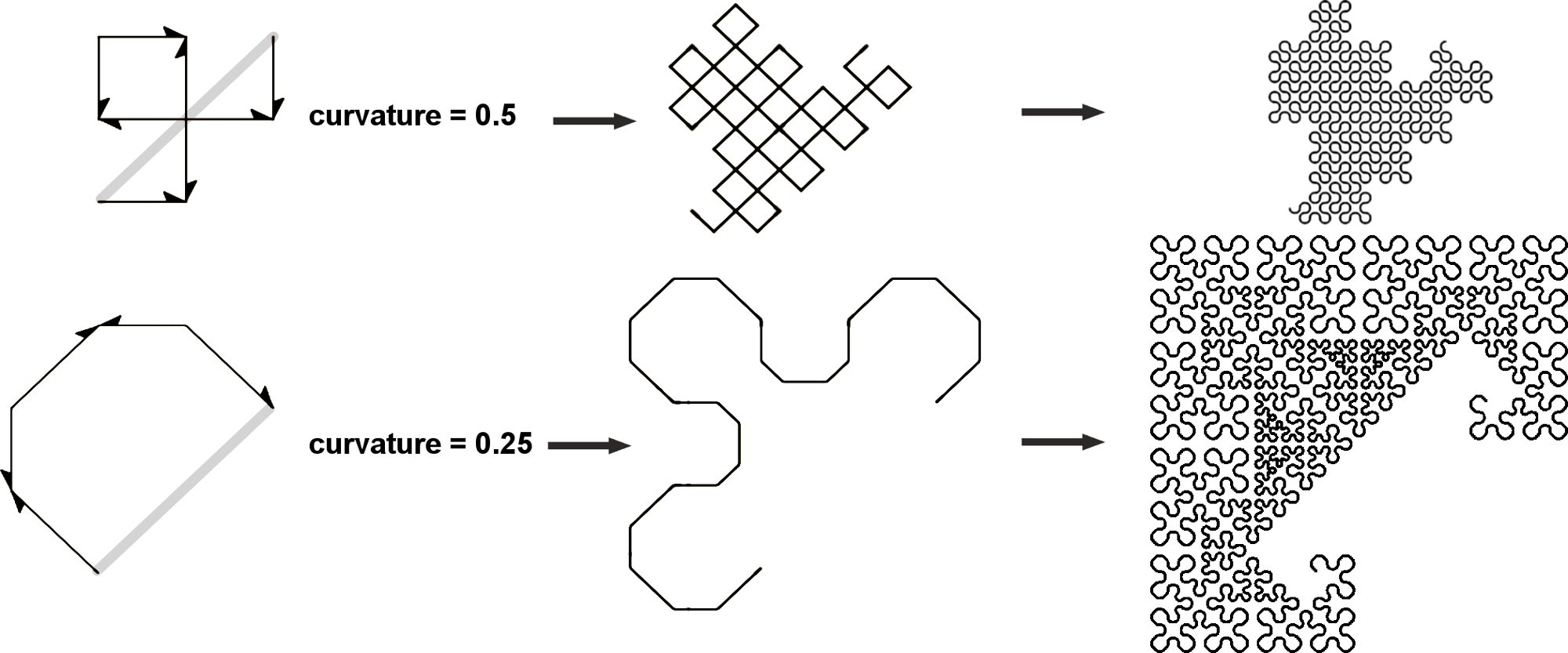Figure 4.34. Differences in curvature in the G(2,2) family Curvature is higher in lattice-fillers (as high as 1/2 in the Gaussian domain [e.g., 5-Dragon], and as high as 2/3 in the Eisenstein domain [e.g., Terdragon]). It appears to be lower in self-avoiding curves. Curves with higher curvature are able to pack more stuff into a smaller space, and thus have smaller areas. However, this is not true in all cases; for example, the two 7-Dragons shown in Figure 4.13 have the same area but different curvatures. In this case, the difference shows up only in the nature of the curve’s sweep—not its area. McKenna observed that plane-filling curves inscribed in equilateral triangles are always self-contacting and never self-avoiding. Conversely, there exist self-avoiding curves with square body shapes . This is related to the observation that it requires more curvature to pack a curve into an equilateral triangle than it does to pack a curve of the same length into a square, assuming both shapes have the same edge length. Point-Symmetrical Curves Many curves have point-symmetry: their shapes remain identical when rotated 180 degrees. This symmetry is apparent in the generator as well as in the associated teragons. Point-symmetric curves appear to be the result of the ordering of the integers in the generator having a palindrome form—being identical when read backwards. There are no 180-rotation transforms in the generators of these curves, since the generators themselves have point symmetry, thus 180-rotating has no effect. Figure 4.35. shows a few such curves. There are no point-symmetrical curves in the G(1,1) family or the G(2,0) family. Are there any other families without point-symmetry? If not, why?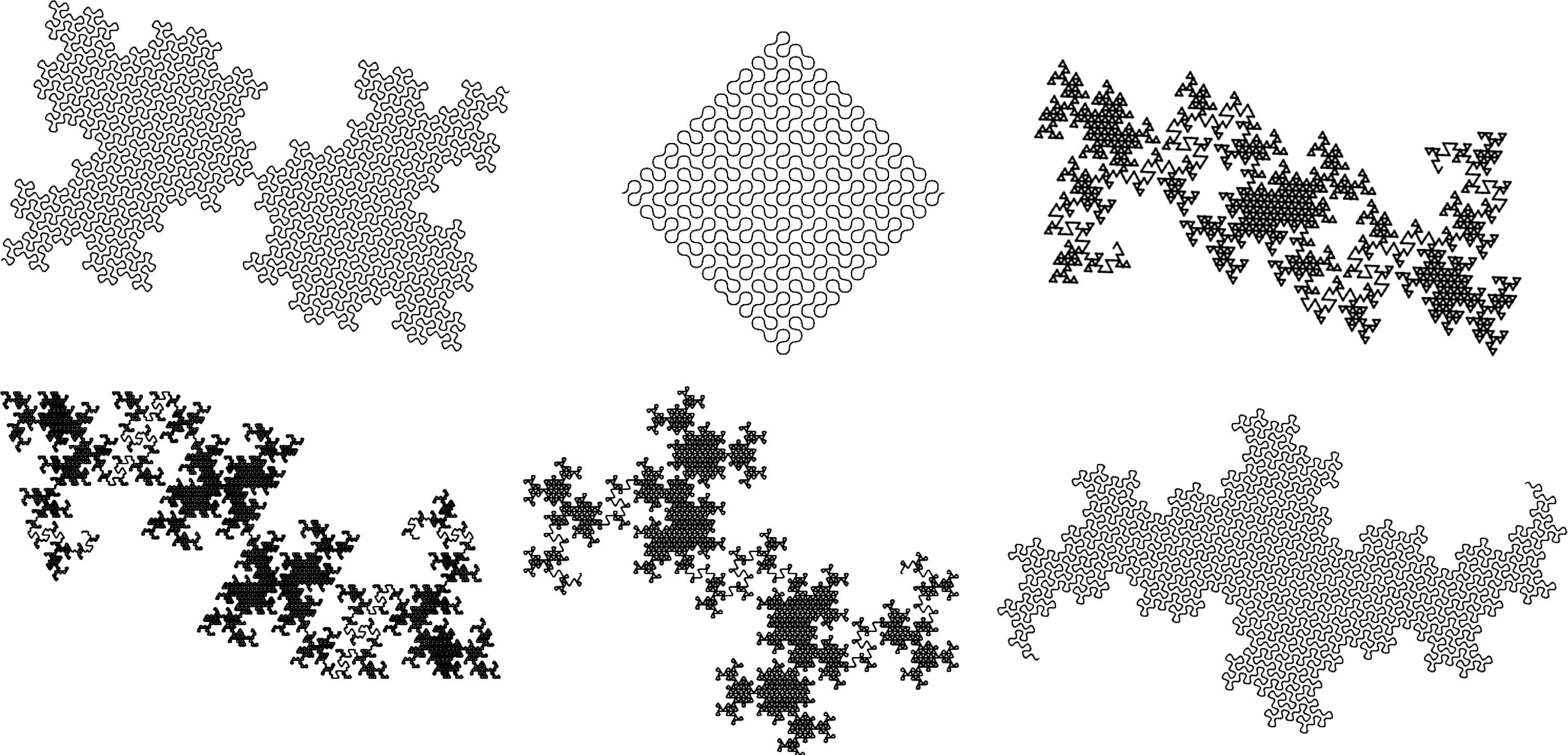Figure 4.35. A few examples of curves with point-symmetry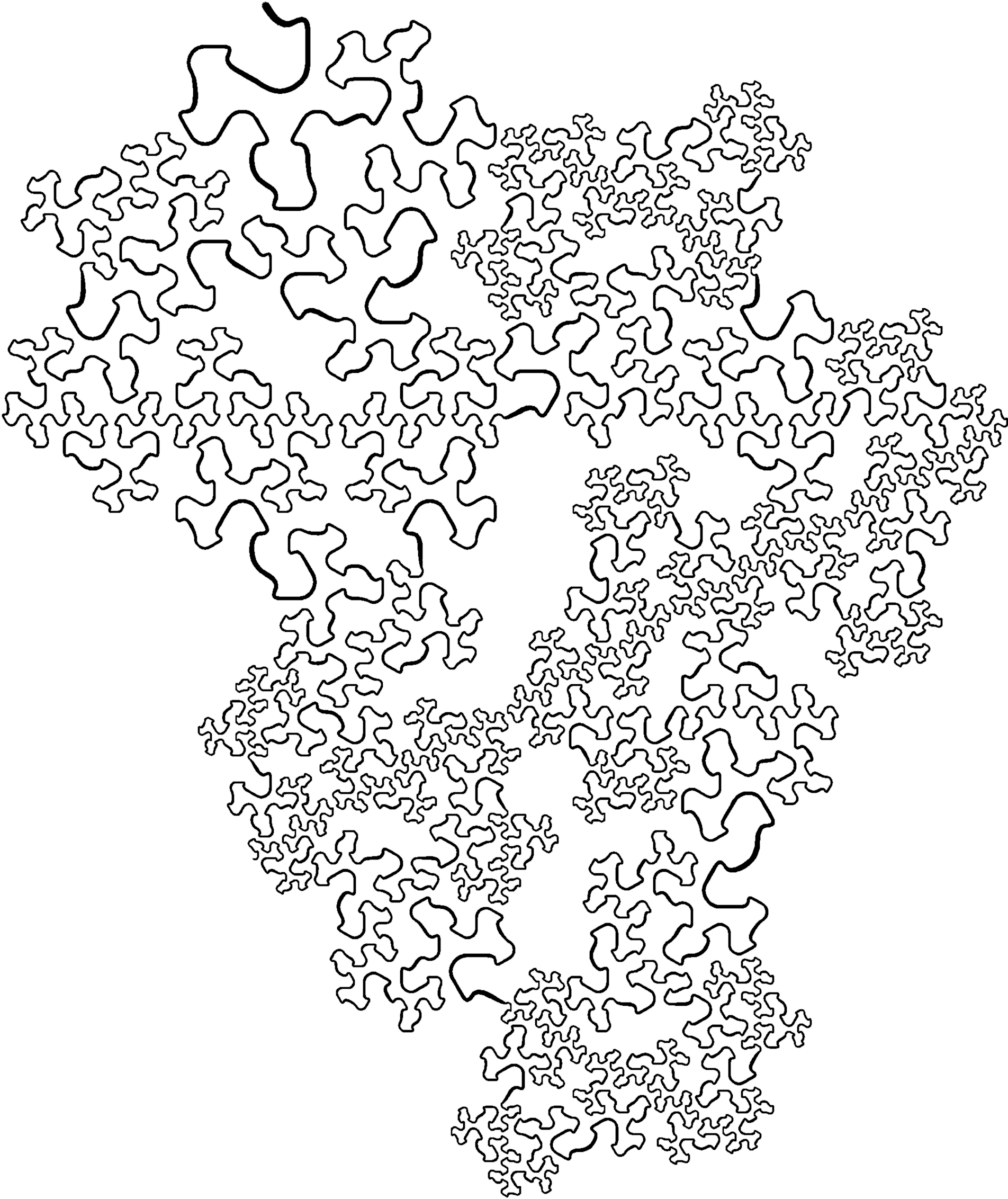Figure 4.36. A stylized curve of the E(3,0) family with dimension < 2The Family Tree of Fractal Curves A taxonomy of plane-filling curves using complex integer lattices by Jeffrey Ventrella 1 Introduction 2 Taxonomy 3 Iteration 4 Morphology 5 Family Resemblances 6 Undiscovered Curiosities 7 References